ń▓ŠÕŹÄÕåģÕ«╣
õĖŗĶĮĮĶĄäµ║É
ķŚ«ńŁö
Õłåń▒╗ńø«ÕĮĢ：ŃĆŖµĘ▒ÕģźńÉåĶ¦Żµ£║ÕÖ©ÕŁ”õ╣ĀŃĆŗµĆ╗ńø«ÕĮĢ ńøĖÕģ│µ¢ćń½Ā： Õ¤║õ║ÄÕå│ńŁ¢µĀæńÜäµ©ĪÕ×ŗ（õĖĆ）Õłåń▒╗µĀæÕÆīÕø×ÕĮÆµĀæ Õ¤║õ║ÄµĀæńÜäµ©ĪÕ×ŗ（õ║ī）：ķøåµłÉÕŁ”õ╣Āõ╣ŗBaggingÕÆīRandom Forest Õ¤║õ║ÄµĀæńÜäµ©ĪÕ×ŗ（õĖē）：ķøåµłÉÕŁ”õ╣Āõ╣ŗGBDTÕÆīXGBoost Õ¤║õ║ÄµĀæńÜäµ©ĪÕ×ŗ（Õøø）：ķÜÅµ£║µŻ«µ×ŚńÜäÕ╗Čõ╝ĖŌĆöŌĆöµĘ▒Õ║”µŻ«µ×Ś（gcForest） Õ¤║õ║ÄµĀæńÜäµ©ĪÕ×ŗ（õ║ö）：õ╗ÄķøČÕ╝ĆÕ¦ŗńö©PythonÕ«×ńÄ░ID3Õå│ńŁ¢µĀæ Õ¤║õ║ÄµĀæńÜäµ©ĪÕ×ŗ（ÕģŁ）：PythonÕ«×ńÄ░CARTÕå│ńŁ¢µĀæÕ╣ČÕł®ńö©Tkinterµ×äÕ╗║GUIÕ»╣Õå│ńŁ¢µĀæĶ┐øĶĪīĶ░āõ╝ś Õ¤║õ║ÄµĀæńÜäµ©ĪÕ×ŗ（õĖā）：RF/XGBoostńŁēń«Śµ│ĢÕ«×ĶĘĄõĖÄÕå│ńŁ¢µĀæScalaÕ«×ĶĘĄńŁē（µØÉµ¢ÖÕćåÕżćõĖŁ）

Õå│ńŁ¢µĀæńö▒ń╗ōńé╣ÕÆīµ£ēÕÉæĶŠ╣ń╗äµłÉŃĆéń╗ōńé╣µ£ēõĖżń¦Źń▒╗Õ×ŗ：Õåģķā©ń╗ōńé╣ÕÆīÕÅČń╗ōńé╣，ÕģČõĖŁÕåģķā©ń╗ōńé╣ĶĪ©ńż║õĖĆõĖ¬ńē╣ÕŠüµł¢Õ▒×µĆ¦，ÕÅČń╗ōńé╣ĶĪ©ńż║õĖĆõĖ¬ń▒╗ŃĆéõĖĆĶł¼ńÜä，õĖĆµŻĄÕå│ńŁ¢µĀæÕīģÕÉ½õĖĆõĖ¬µĀ╣ń╗ōńé╣ŃĆüĶŗźÕ╣▓õĖ¬Õåģķā©ń╗ōńé╣ÕÆīĶŗźÕ╣▓õĖ¬ÕÅČń╗ōńé╣ŃĆéÕÅČń╗ōńé╣Õ»╣Õ║öõ║ÄÕå│ńŁ¢ń╗ōµ×£，ÕģČõ╗¢µ»ÅõĖ¬ń╗ōńé╣ÕłÖÕ»╣Õ║öõ║ÄõĖĆõĖ¬Õ▒×µĆ¦µĄŗĶ»ĢŃĆéµ»ÅõĖ¬ń╗ōńé╣ÕīģÕÉ½ńÜäµĀĘµ£¼ķøåÕÉłµĀ╣µŹ«Õ▒×µĆ¦µĄŗĶ»ĢńÜäń╗ōµ×£Ķó½ÕłÆÕłåÕł░ÕŁÉń╗ōńé╣õĖŁ，µĀ╣ń╗ōńé╣ÕīģÕÉ½µĀĘµ£¼Õģ©ķøå，õ╗ÄµĀ╣ń╗ōńé╣Õł░µ»ÅõĖ¬ÕÅČń╗ōńé╣ńÜäĶĘ»ÕŠäÕ»╣Õ║öõ║åõĖĆõĖ¬ÕłżÕ«ÜµĄŗĶ»ĢÕ║ÅÕłŚŃĆéÕ£©õĖŗÕøŠõĖŁ，Õ£åÕÆīµ¢╣µĪåÕłåÕł½ĶĪ©ńż║Õåģķā©ń╗ōńé╣ÕÆīÕÅČń╗ōńé╣ŃĆéÕå│ńŁ¢µĀæÕŁ”õ╣ĀńÜäńø«ńÜäµś»õĖ║õ║åõ║¦ńö¤õĖĆµŻĄµ│øÕī¢ĶāĮÕŖøÕ╝║，ÕŹ│ÕżäńÉåµ£¬Ķ¦üńż║õŠŗĶāĮÕŖøÕ╝║ńÜäÕå│ńŁ¢µĀæŃĆéÕłåń▒╗µĀæ
ÕüćĶ«Šń╗ÖÕ«ÜĶ«Łń╗āµĢ░µŹ«ķøå：

D

=

{

(

x

1

,

y

1

)

,

(

x

2

,

y

2

)

,

.

.

.

,

(

x

N

,

y

N

)

}

D=\{(x_1, y_1), (x_2, y_2), ..., (x_N, y_N)\}

ÕģČõĖŁ，

x

i

=

(

x

i

(

1

)

,

x

i

(

2

)

,

.

.

.

,

x

i

(

n

)

)

T

,

x_i=(x_i^{(1)}, x_i^{(2)}, ..., x_i^{(n)})^T,

õĖ║ĶŠōÕģźÕ«×õŠŗ，ÕŹ│ńē╣ÕŠüÕÉæķćÅ，

n

n

õĖ║ńē╣ÕŠüõĖ¬µĢ░，

i

=

1

，

2

ŌĆ”

，

N

i=1，2ŌĆ”，N

，

N

N

õĖ║µĀĘµ£¼Õ«╣ķćÅ，

y

i

Ōłł

{

1

,

2

,

.

.

.

,

K

}

y_i \in \{ 1, 2, ..., K\}

Õå│ńŁ¢µĀæÕłåń▒╗ń«Śµ│Ģ ĶŠōÕģź：

Ķ«Łń╗āķøå：

D

=

(

x

1

,

y

1

)

,

(

x

2

,

y

2

)

,

Ōŗ»
ŌĆē

,

(

x

N

,

y

N

)

D = {(x_1, y_1), (x_2, y_2), \cdots, (x_N, y_N)}

Õ▒×µĆ¦ķøå：

A

=

a

1

,

a

2

,

Ōŗ»
ŌĆē

,

a

n

A = {a_1, a_2, \cdots, a_n}

ÕćĮµĢ░

T

r

e

e

G

e

n

e

r

a

t

e

(

D

,

A

)

TreeGenerate(D, A)

ĶŠōÕć║：

õ╗źnodeõĖ║µĀ╣ĶŖéńé╣ńÜäÕå│ńŁ¢µĀæ ń«Śµ│Ģ： （ 1 ） ńö¤µłÉń╗ōńé╣µĀ╣node （ 2 ） if

D

D

C

k

C_k

then （ 3 ）

Õ░ånodeµĀćĶ«░õĖ║

C

k

C_k

ń▒╗ÕÅČń╗ōńé╣ （ 4 ）

return （ 5 ） end if （ 6 ） if

A

=

Ōłģ

A = \varnothing

OR

D

D

A

A

õĖŖÕÅ¢ÕĆ╝ńøĖÕÉī then （ 7 ）

Õ░ånodeµĀćĶ«░õĖ║ÕÅČń╗ōńé╣，ÕģČń▒╗Õł½µĀćĶ«░õĖ║

D

D

õĖŁµĀĘµ£¼µĢ░µ£ĆÕżÜńÜäń▒╗ （ 8 ）

return （ 9 ）end if （10）õ╗Ä

A

A

õĖŁķĆēµŗ®µ£Ćõ╝śÕłÆÕłåÕ▒×µĆ¦

a

ŌłŚ

a_*

（11）for

a

ŌłŚ

a_*

ńÜäµ»ÅõĖĆõĖ¬ÕĆ╝

a

ŌłŚ

v

a_*^v

do （12）

õĖ║nodeńö¤µłÉõĖĆõĖ¬Õłåµö»：õ╗ż

D

v

D_v

D

D

a

ŌłŚ

a_*

õĖŖÕÅ¢ÕĆ╝õĖ║

a

ŌłŚ

v

a_*^v

ńÜäµĀĘµ£¼ÕŁÉķøå （13）

if

D

v

D_v

õĖ║ń®║ then （14）

Õ░åÕłåµö»ń╗ōńé╣µĀćĶ«░õĖ║ÕÅČń╗ōńé╣，ÕģČń▒╗Õł½µĀćĶ«░õĖ║

D

D

õĖŁµĀĘµ£¼µ£ĆÕżÜńÜäń▒╗ （15）

return （16）

else （17）

õ╗ź

T

r

e

e

G

e

n

e

r

a

t

e

(

D

v

,

A

ŌłÆ

{

a

ŌłŚ

}

)

TreeGenerate(D_v, A - \{a_*\})

õĖ║Õłåµö»ń╗ōńé╣ （18）

end if （19）end for

Õå│ńŁ¢µĀæõĖÄif-thenĶ¦äÕłÖ
Õå│ńŁ¢µĀæõĖÄµØĪõ╗Čµ”éńÄćÕłåÕĖā

X

X

Y

Y

P

（

Y

ŌłŻ

X

）

P（Y|X）

ŃĆé

X

X

ÕÅ¢ÕĆ╝õ║Äń╗ÖÕ«ÜÕłÆÕłåõĖŗÕŹĢÕģāńÜäķøåÕÉł，

Y

Y

ÕÅ¢ÕĆ╝õ║Äń▒╗ńÜäķøåÕÉłŃĆéÕÉäÕÅČń╗ōńé╣（ÕŹĢÕģā）õĖŖńÜäµØĪõ╗Čµ”éńÄćÕŠĆÕŠĆÕüÅÕÉæµ¤ÉõĖĆõĖ¬ń▒╗，ÕŹ│Õ▒×õ║Äµ¤ÉõĖĆń▒╗ńÜäµ”éńÄćĶŠāÕż¦ŃĆéÕå│ńŁ¢µĀæÕłåń▒╗µŚČÕ░åĶ»źń╗ōńé╣ńÜäÕ«×õŠŗÕ╝║ĶĪīÕłåÕł░µØĪõ╗Čµ”éńÄćÕż¦ńÜäķéŻõĖĆń▒╗ÕÄ╗ŃĆé
Õå│ńŁ¢µĀæńÜäõ╝śń╝║ńé╣
ńē╣ÕŠüķĆēµŗ®
õ┐Īµü»Õó×ńøŖ

X

X

µś»õĖĆõĖ¬ÕÅ¢µ£ēķÖÉõĖ¬ÕĆ╝ńÜäń”╗µĢŻķÜÅµ£║ÕÅśķćÅ，ÕģČµ”éńÄćÕłåÕĖāõĖ║:

P

(

X

=

x

i

)

=

p

i

,

i

=

1

,

2

,

Ōŗ»
ŌĆē

,

n

P(X = x_i) = p_i, i = 1, 2, \cdots, n

ÕłÖķÜÅµ£║ÕÅśķćÅ

X

X

ńÜäńåĄÕ«Üõ╣ēõĖ║：

H

(

X

)

=

ŌłÆ

Ōłæ

i

=

1

n

p

i

log

ŌüĪ

p

i

H(X) = -\sum_{i = 1}^n p_i \log p_i

p

i

=

0

p_i = 0

，ÕłÖÕ«Üõ╣ē

p

i

log

ŌüĪ

p

i

=

0

p_i \log p_i = 0

ŃĆéķĆÜÕĖĖ，õĖŖÕ╝ÅõĖŁńÜäÕ»╣µĢ░õ╗ź

2

2

õĖ║Õ║Ģµł¢õ╗ź

e

e

õĖ║Õ║Ģ（Ķć¬ńäČÕ»╣µĢ░），Ķ┐ÖµŚČńåĄńÜäÕŹĢõĮŹÕłåÕł½ń¦░õĮ£µ»öńē╣（bit）µł¢ń║│ńē╣（nat）.ńö▒Õ«Üõ╣ēÕÅ»ń¤ź，ńåĄÕÅ¬õŠØĶĄ¢õ║Ä

X

X

ńÜäÕłåÕĖā，ĶĆīõĖÄ

X

X

ńÜäÕÅ¢ÕĆ╝µŚĀÕģ│，µēĆõ╗źõ╣¤ÕÅ»Õ░å

X

X

ńÜäńåĄĶ«░õĮ£

H

(

p

)

H(p)

，ÕŹ│:

H

(

p

)

=

ŌłÆ

Ōłæ

i

=

1

n

p

i

log

ŌüĪ

p

i

H(p) = -\sum_{i = 1}^n p_i \log p_i

ńö▒µŁżÕÅ»Ķ¦ü，ńåĄĶČŖÕż¦，ķÜÅµ£║ÕÅśķćÅńÜäõĖŹńĪ«Õ«ÜµĆ¦Õ░▒ĶČŖÕż¦ŃĆéõ╗ÄńåĄńÜäÕ«Üõ╣ēÕÅ»ķ¬īĶ»ü

0

Ōēż

H

(

p

)

Ōēż

log

ŌüĪ

n

0 \leq H(p) \leq \log n

ÕĮōķÜÅµ£║ÕÅśķćÅÕÅ¬ÕÅ¢õĖżõĖ¬ÕĆ╝，õŠŗÕ”é1，0µŚČ，ÕŹ│

X

X

ńÜäÕłåÕĖāõĖ║:

P

(

X

=

1

)

=

p

,

P

(

X

=

0

)

=

1

ŌłÆ

p

,

0

Ōēż

p

Ōēż

1

P(X = 1) = p,\quad P(X = 0) = 1-p, \quad 0ŌēżpŌēż1

ÕģČńåĄõĖ║：

H

(

p

)

=

ŌłÆ

p

log

ŌüĪ

2

p

ŌłÆ

(

1

ŌłÆ

p

)

log

ŌüĪ

2

(

1

ŌłÆ

p

)

H(p) = -p \log_2 p - (1 - p)\log_2 (1 - p)

Ķ┐ÖµŚČ，ńåĄ

H

(

p

)

H(p)

ķÜÅµ”éńÄć

p

p

ÕÅśÕī¢ńÜäµø▓ń║┐Õ”éõĖŗÕøŠµēĆńż║（ÕŹĢõĮŹõĖ║µ»öńē╣）：ÕĮō

p

=

0

p = 0

µł¢

p

=

1

p = 1

µŚČ

H

(

p

)

=

0

H(p) = 0

p

=

0.5

p = 0.5

µŚČ，

H

(

p

)

=

1

H(p) = 1

，ńåĄÕÅ¢ÕĆ╝µ£ĆÕż¦，ķÜÅµ£║ÕÅśķćÅõĖŹńĪ«Õ«ÜµĆ¦µ£ĆÕż¦ŃĆé
Ķ«Šµ£ēķÜÅµ£║ÕÅśķćÅ

(

X

,

Y

)

(X, Y)

，ÕģČĶüöÕÉłµ”éńÄćÕłåÕĖāõĖ║：

P

(

X

=

x

i

,

Y

=

y

i

)

=

p

i

j

{

i

=

1

,

2

,

Ōŗ»
ŌĆē

,

n

j

=

1

,

2

,

Ōŗ»
ŌĆē

,

m

P(X = x_i, Y = y_i) = p_{ij} \quad \begin{cases} i = 1, 2, \cdots, n \\ j = 1, 2, \cdots, m \end{cases}

µØĪõ╗ČńåĄ

H

(

Y

ŌłŻ

X

)

H(Y|X)

X

X

ńÜäµØĪõ╗ČõĖŗķÜÅµ£║ÕÅśķćÅ

Y

Y

ńÜäõĖŹńĪ«Õ«ÜµĆ¦ŃĆéķÜÅµ£║ÕÅśķćÅ

X

X

ń╗ÖÕ«ÜńÜäµØĪõ╗ČõĖŗķÜÅµ£║ÕÅśķćÅ

Y

Y

ńÜäµØĪõ╗ČńåĄ（conditional entropy）

H

(

Y

ŌłŻ

X

)

H(Y|X)

，Õ«Üõ╣ēõĖ║

X

X

ń╗ÖÕ«ÜµØĪõ╗ČõĖŗ

Y

Y

ńÜäµØĪõ╗Čµ”éńÄćÕłåÕĖāńÜäńåĄÕ»╣

X

X

ńÜäµĢ░ÕŁ”µ£¤µ£ø：

H

(

Y

ŌłŻ

X

)

=

Ōłæ

i

=

1

n

p

i

H

(

Y

ŌłŻ

X

=

x

i

)

H(Y|X) = \sum_{i = 1}^n p_iH(Y|X = x_i)

ÕģČõĖŁ，

p

i

=

P

(

X

=

x

i

)

,

i

=

1

,

2

,

Ōŗ»
ŌĆē

,

n

p_i = P(X = x_i), i = 1, 2, \cdots, n

ŃĆé
ÕĮōńåĄÕÆīµØĪõ╗ČńåĄõĖŁńÜäµ”éńÄćńö▒µĢ░µŹ«õ╝░Ķ«Ī（Õ”éµ×üÕż¦õ╝╝ńäČõ╝░Ķ«Ī）ÕŠŚÕł░µŚČ，µēĆÕ»╣Õ║öńÜäńåĄõĖÄµØĪõ╗ČńåĄÕłåÕł½ń¦░õĖ║ń╗Åķ¬īńåĄ（empirical entropy）ÕÆīń╗Åķ¬īµØĪõ╗ČńåĄ（empirical conditional entropy）ŃĆéµŁżµŚČ，Õ”éµ×£µ£ē0µ”éńÄć，õ╗ż

0

log

ŌüĪ

0

=

0

0\log0 = 0

ŃĆé

X

X

ńÜäõ┐Īµü»ĶĆīõĮ┐ÕŠŚń▒╗

Y

Y

a

ŌłŚ

a_*

Õ»╣Ķ«Łń╗āµĢ░µŹ«ķøå

D

D

ńÜäõ┐Īµü»Õó×ńøŖ

g

(

D

,

a

ŌłŚ

)

g(D, a_*)

，Õ«Üõ╣ēõĖ║ķøåÕÉł

D

D

ńÜäń╗Åķ¬īńåĄ

H

(

D

)

H(D)

õĖÄńē╣ÕŠü

a

ŌłŚ

a_*

ń╗ÖÕ«ÜµØĪõ╗ČõĖŗ

D

D

ńÜäń╗Åķ¬īµØĪõ╗ČńåĄ

H

(

D

ŌłŻ

a

ŌłŚ

)

H(D|a_*)

õ╣ŗÕĘ«，ÕŹ│：

g

(

D

,

a

ŌłŚ

)

=

H

(

D

)

ŌłÆ

H

(

D

ŌłŻ

a

ŌłŚ

)

g(D, a_*) = H(D) - H(D|a_*)

õĖĆĶł¼Õ£░，ńåĄ

H

(

Y

)

H(Y)

õĖÄµØĪõ╗ČńåĄ

H

(

Y

ŌłŻ

X

)

H(Y|X)

õ╣ŗÕĘ«ń¦░õĖ║õ║Æõ┐Īµü»（mutual information）ŃĆéÕå│ńŁ¢µĀæÕŁ”õ╣ĀõĖŁńÜäõ┐Īµü»Õó×ńøŖńŁēõ╗Ęõ║ÄĶ«Łń╗āµĢ░µŹ«ķøåõĖŁń▒╗õĖÄńē╣ÕŠüńÜäõ║Æõ┐Īµü»ŃĆé

D

D

ÕÆīńē╣ÕŠü

a

ŌłŚ

a_*

，ń╗Åķ¬īńåĄ

H

(

D

)

H(D)

D

D

Ķ┐øĶĪīÕłåń▒╗ńÜäõĖŹńĪ«Õ«ÜµĆ¦ŃĆéĶĆīń╗Åķ¬īµØĪõ╗ČńåĄ

H

(

D

ŌłŻ

a

ŌłŚ

)

H(D|a_*)

a

ŌłŚ

a_*

ń╗ÖÕ«ÜńÜäµØĪõ╗ČõĖŗÕ»╣µĢ░µŹ«ķøå

D

D

a

ŌłŚ

a_*

ĶĆīõĮ┐ÕŠŚÕ»╣µĢ░µŹ«ķøå

D

D

D

D

µĀ╣µŹ«õ┐Īµü»Õó×ńøŖÕćåÕłÖńÜäńē╣ÕŠüķĆēµŗ®µ¢╣µ│Ģ：Õ»╣Ķ«Łń╗āµĢ░µŹ«ķøå（µł¢ÕŁÉķøå）

D

D

，Ķ«Īń«ŚÕģČµ»ÅõĖ¬ńē╣ÕŠüńÜäõ┐Īµü»Õó×ńøŖ，Õ╣Čµ»öĶŠāÕ«āõ╗¼ńÜäÕż¦Õ░Å，ķĆēµŗ®õ┐Īµü»Õó×ńøŖµ£ĆÕż¦ńÜäńē╣ÕŠüŃĆé
Ķ«ŠĶ«Łń╗āµĢ░µŹ«ķøåõĖ║

D

D

，

ŌłŻ

D

ŌłŻ

|D|

K

K

õĖ¬ń▒╗

C

k

C_k

，

k

=

1

,

2

,

Ōŗ»
ŌĆē

,

K

k=1, 2, \cdots, K

，

ŌłŻ

C

k

ŌłŻ

|C_k|

õĖ║Õ▒×õ║Äń▒╗

C

k

C_k

ńÜäµĀĘµ£¼õĖ¬µĢ░，

Ōłæ

k

=

1

K

ŌłŻ

C

k

ŌłŻ

=

ŌłŻ

D

ŌłŻ

\sum_{k=1}^K |C_k| = |D|

ŃĆéĶ«Šńē╣ÕŠü

a

ŌłŚ

a_*

µ£ē

V

V

õĖ¬õĖŹÕÉīńÜäÕÅ¢ÕĆ╝

{

a

ŌłŚ

1

,

a

ŌłŚ

2

,

Ōŗ»
ŌĆē

,

a

ŌłŚ

V

}

\{ a_*^1, a_*^2, \cdots, a_*^V\}

，µĀ╣µŹ«ńē╣ÕŠü

a

ŌłŚ

a_*

ńÜäÕÅ¢ÕĆ╝Õ░å

D

D

ÕłÆÕłåõĖ║

V

V

õĖ¬ÕŁÉķøå

D

1

,

D

2

,

Ōŗ»
ŌĆē

,

D

V

D_1, D_2, \cdots, D_V

，

ŌłŻ

D

t

ŌłŻ

|D_t|

õĖ║

D

t

D_t

ńÜäµĀĘµ£¼õĖ¬µĢ░，

Ōłæ

i

=

1

n

ŌłŻ

D

t

ŌłŻ

=

ŌłŻ

D

ŌłŻ

\sum_{i=1}^n|D_t|=|D|

ŃĆéĶ«░ÕŁÉķøå

D

i

D_i

õĖŁÕ▒×õ║Äń▒╗

C

k

C_k

ńÜäµĀĘµ£¼ńÜäķøåÕÉłõĖ║

D

i

k

D_{ik}

ŃĆéÕŹ│

D

i

k

=

D

i

Ōł®

C

k

D_{ik} = D_i \cap C_k

，

ŌłŻ

D

i

k

ŌłŻ

|D_{ik}|

õĖ║

D

i

k

D_{ik}

ńÜäµĀĘµ£¼õĖ¬µĢ░ŃĆéõ║Äµś»Ķ«Īń«Śõ┐Īµü»Õó×ńøŖńÜäµ¢╣µ│ĢÕ”éõĖŗ：

õ┐Īµü»Õó×ńøŖ ĶŠōÕģź：Ķ«Łń╗āµĢ░µŹ«ķøå

D

D

ÕÆīńē╣ÕŠü

a

ŌłŚ

a_*

ĶŠōÕć║：ńē╣ÕŠü

a

ŌłŚ

a_*

Õ»╣Ķ«Łń╗āµĢ░µŹ«ķøå

D

D

ńÜäõ┐Īµü»Õó×ńøŖ

g

(

D

,

a

ŌłŚ

)

g(D, a_*)

1.Ķ«Īń«ŚµĢ░µŹ«ķøå

D

D

ńÜäń╗Åķ¬īńåĄ

H

(

D

)

H(D)

：

H

(

D

)

=

ŌłÆ

Ōłæ

k

=

1

K

C

k

D

log

ŌüĪ

2

C

k

D

H(D) = -\sum_{k=1}^K \frac{C_k}{D}\log_2\frac{C_k}{D}

2.Ķ«Īń«Śńē╣ÕŠü

A

A

Õ»╣µĢ░µŹ«ķøå

D

D

ńÜäń╗Åķ¬īµØĪõ╗ČńåĄ

H

(

D

ŌłŻ

A

)

H(D|A)

：

H

(

D

ŌłŻ

A

)

=

Ōłæ

i

=

1

n

ŌłŻ

D

i

ŌłŻ

D

H

(

D

i

)

=

ŌłÆ

Ōłæ

i

=

1

n

ŌłŻ

D

i

ŌłŻ

D

Ōłæ

k

=

1

K

D

i

k

D

i

log

ŌüĪ

2

D

i

k

D

i

H(D|A) = \sum_{i=1}^n\frac{|D_i|}{D}H(D_i)=-\sum_{i=1}^n\frac{|D_i|}{D}\sum_{k=1}^K\frac{D_{ik}}{D_i}\log_2\frac{D_{ik}}{D_i}

3.Ķ«Īń«Śõ┐Īµü»Õó×ńøŖ：

g

(

D

,

a

ŌłŚ

)

=

H

(

D

)

ŌłÆ

H

(

D

ŌłŻ

a

ŌłŚ

)

g(D, a_*) = H(D) - H(D|a_*)

a

ŌłŚ

a_*

a

ŌłŚ

=

arg┬Ā

max

ŌüĪ

a

Ōłł

A

g

(

D

,

a

)

a_* = \text{arg}\ \max_{a \in A}g(D, a)

ķĆēµŗ®µ£Ćõ╝śÕłÆÕłåÕ▒×µĆ¦ŃĆéĶæŚÕÉŹńÜäID3Õå│ńŁ¢µĀæÕŁ”õ╣Āń«Śµ│ĢÕ░▒µś»õ╗źõ┐Īµü»Õó×ńøŖõĖ║ÕćåÕłÖµØźķĆēµŗ®ÕłÆÕłåÕ▒×µĆ¦ŃĆé

ID3ń«Śµ│Ģ ĶŠōÕģź：Ķ«Łń╗āµĢ░µŹ«ķøå

D

D

，ńē╣ÕŠüķøå

A

A

，ķśłÕĆ╝

ŽĄ

\epsilon

ĶŠōÕć║：Õå│ńŁ¢µĀæ

T

T

1.Ķŗź

D

D

õĖŁµēĆµ£ēÕ«×õŠŗÕ▒×õ║ÄÕÉīõĖĆń▒╗

C

k

C_k

，ÕłÖ

T

T

õĖ║ÕŹĢń╗ōńé╣µĀæ，Õ╣ČÕ░åń▒╗

C

k

C_k

õĮ£õĖ║Ķ»źń╗ōńé╣ńÜäń▒╗µĀćĶ«░，Ķ┐öÕø×Õå│ńŁ¢µĀæ

T

T

2.Ķŗź

A

=

Ōłģ

A = \varnothing

，ÕłÖ

T

T

õĖ║ÕŹĢń╗ōńé╣µĀæ，Õ╣ČÕ░å

D

D

õĖŁÕ«×õŠŗµĢ░µ£ĆÕż¦ńÜäń▒╗

C

k

C_k

，õĮ£õĖ║Ķ»źń╗ōńé╣ńÜäń▒╗µĀćĶ«░，Ķ┐öÕø×Õå│ńŁ¢µĀæ

T

T

3.ÕÉ”ÕłÖ，Ķ«Īń«Ś

A

A

õĖŁÕÉäńē╣ÕŠüÕ»╣

D

D

ńÜäõ┐Īµü»Õó×ńøŖ，ķĆēµŗ®õ┐Īµü»Õó×ńøŖµ£ĆÕż¦ńÜäńē╣ÕŠü

a

ŌłŚ

a_*

4.Õ”éµ×£

a

ŌłŚ

a_*

ńÜäõ┐Īµü»Õó×ńøŖÕ░Åõ║ÄķśłÕĆ╝

ŽĄ

\epsilon

，ÕłÖńĮ«

T

T

õĖ║ÕŹĢń╗ōńé╣µĀæ，Õ╣ČÕ░å

D

D

õĖŁÕ«×õŠŗµĢ░µ£ĆÕż¦ńÜäń▒╗

C

k

C_k

õĮ£õĖ║Ķ»źń╗ōńé╣ńÜäń▒╗µĀćĶ«░，Ķ┐öÕø×Õå│ńŁ¢µĀæ

T

T

5.ÕÉ”ÕłÖ，Õ»╣

a

ŌłŚ

a_*

ńÜäµ»ÅõĖĆÕÅ»ĶāĮÕĆ╝

a

ŌłŚ

v

a_*^v

，õŠØ

a

ŌłŚ

=

a

ŌłŚ

v

a_* = a_*^v

Õ░å

D

D

ÕłåÕē▓õĖ║ĶŗźÕ╣▓ķØ×ń®║ÕŁÉķøå

D

v

D_v

，Õ░å

D

v

D_v

õĖŁÕ«×õŠŗµĢ░µ£ĆÕż¦ńÜäń▒╗õĮ£õĖ║µĀćĶ«░，µ×äÕ╗║ÕŁÉń╗ōńé╣，ńö▒ń╗ōńé╣ÕÅŖÕģČÕŁÉń╗ōńé╣µ×äµłÉÕå│ńŁ¢µĀæ

T

T

，Ķ┐öÕø×Õå│ńŁ¢µĀæ

T

T

6.Õ»╣ń¼¼

v

v

õĖ¬ÕŁÉń╗ōńé╣，õ╗ź

D

v

D_v

õĖ║Ķ«Łń╗āķøå，õ╗ź

A

ŌłÆ

{

a

ŌłŚ

}

A - \{a_*\}

T

v

T_v

，Õ╣ČĶ┐öÕø×ÕŁÉµĀæ

T

v

T_v

õ┐Īµü»Õó×ńøŖńÄć

a

ŌłŚ

a_*

Õ»╣Ķ«Łń╗āµĢ░µŹ«ķøå

D

D

ńÜäõ┐Īµü»Õó×ńøŖńÄć

g

g

(

D

,

a

ŌłŚ

)

g_g(D, a_*)

Õ«Üõ╣ēõĖ║ÕģČõ┐Īµü»Õó×ńøŖ

g

(

D

,

a

ŌłŚ

)

g(D, a_*)

õĖÄĶ«Łń╗āµĢ░µŹ«ķøå

D

D

ńÜäń╗Åķ¬īńåĄ

H

(

D

)

H(D)

õ╣ŗµ»ö：

g

g

(

D

,

a

ŌłŚ

)

=

g

(

D

,

a

ŌłŚ

)

H

(

D

)

g_g(D, a_*) = \frac{g(D, a_*)}{H(D)}

C4.5ń«Śµ│Ģ ĶŠōÕģź：Ķ«Łń╗āµĢ░µŹ«ķøå

D

D

，ńē╣ÕŠüķøå

A

A

，õ┐Īµü»Õó×ńøŖńÄćķśłÕĆ╝

ŽĄ

\epsilon

，õ┐Īµü»Õó×ńøŖķśłÕĆ╝

╬▒

\alpha

ĶŠōÕć║：Õå│ńŁ¢µĀæ

T

T

1.Ķŗź

D

D

õĖŁµēĆµ£ēÕ«×õŠŗÕ▒×õ║ÄÕÉīõĖĆń▒╗

C

k

C_k

，ÕłÖ

T

T

õĖ║ÕŹĢń╗ōńé╣µĀæ，Õ╣ČÕ░åń▒╗

C

k

C_k

õĮ£õĖ║Ķ»źń╗ōńé╣ńÜäń▒╗µĀćĶ«░，Ķ┐öÕø×Õå│ńŁ¢µĀæ

T

T

2.Ķŗź

A

=

Ōłģ

A = \varnothing

，ÕłÖ

T

T

õĖ║ÕŹĢń╗ōńé╣µĀæ，Õ╣ČÕ░å

D

D

õĖŁÕ«×õŠŗµĢ░µ£ĆÕż¦ńÜäń▒╗

C

k

C_k

，õĮ£õĖ║Ķ»źń╗ōńé╣ńÜäń▒╗µĀćĶ«░，Ķ┐öÕø×Õå│ńŁ¢µĀæ

T

T

3.ÕÉ”ÕłÖ，Ķ«Īń«Ś

A

A

õĖŁÕÉäńē╣ÕŠüÕ»╣

D

D

╬▒

\alpha

ńÜäńē╣ÕŠüõĖŁķĆēµŗ®õ┐Īµü»Õó×ńøŖńÄćµ£ĆÕż¦ńÜäńē╣ÕŠü

a

ŌłŚ

a_*

4.Õ”éµ×£

a

ŌłŚ

a_*

ńÜäõ┐Īµü»Õó×ńøŖńÄćÕ░Åõ║ÄķśłÕĆ╝

ŽĄ

\epsilon

，ÕłÖńĮ«

T

T

õĖ║ÕŹĢń╗ōńé╣µĀæ，Õ╣ČÕ░å

D

D

õĖŁÕ«×õŠŗµĢ░µ£ĆÕż¦ńÜäń▒╗

C

k

C_k

õĮ£õĖ║Ķ»źń╗ōńé╣ńÜäń▒╗µĀćĶ«░，Ķ┐öÕø×Õå│ńŁ¢µĀæ

T

T

5.ÕÉ”ÕłÖ，Õ»╣

a

ŌłŚ

a_*

ńÜäµ»ÅõĖĆÕÅ»ĶāĮÕĆ╝

a

ŌłŚ

v

a_*^v

，õŠØ

a

ŌłŚ

=

a

ŌłŚ

v

a_* = a_*^v

Õ░å

D

D

ÕłåÕē▓õĖ║ĶŗźÕ╣▓ķØ×ń®║ÕŁÉķøå

D

v

D_v

，Õ░å

D

v

D_v

õĖŁÕ«×õŠŗµĢ░µ£ĆÕż¦ńÜäń▒╗õĮ£õĖ║µĀćĶ«░，µ×äÕ╗║ÕŁÉń╗ōńé╣，ńö▒ń╗ōńé╣ÕÅŖÕģČÕŁÉń╗ōńé╣µ×äµłÉÕå│ńŁ¢µĀæ

T

T

，Ķ┐öÕø×Õå│ńŁ¢µĀæ

T

T

6.Õ»╣ń¼¼

v

v

õĖ¬ÕŁÉń╗ōńé╣，õ╗ź

D

v

D_v

õĖ║Ķ«Łń╗āķøå，õ╗ź

A

ŌłÆ

{

a

ŌłŚ

}

A - \{a_*\}

T

v

T_v

，Õ╣ČĶ┐öÕø×ÕŁÉµĀæ

T

v

T_v

Ķ┐×ń╗ŁÕĆ╝ÕżäńÉå
ń╗ÖÕ«ÜµĀĘµ£¼ķøå

D

D

ÕÆīĶ┐×ń╗ŁÕ▒×µĆ¦

a

a

，ÕüćÕ«Ü

a

a

D

D

õĖŖÕć║ńÄ░õ║å

n

n

õĖ¬õĖŹÕÉīńÜäÕÅ¢ÕĆ╝，Õ░åĶ┐Öõ║øÕĆ╝õ╗ÄÕ░ÅÕł░Õż¦Ķ┐øĶĪīµÄÆÕ║Å，Ķ«░õĖ║

{

a

1

,

a

2

,

a

3

,

Ōŗ»
ŌĆē

,

a

n

}

\{a_1, a_2, a_3, \cdots, a_n\}

ŃĆéÕ¤║õ║ÄÕłÆÕłåńé╣

t

t

ÕÅ»Õ░å

D

D

ÕłåõĖ║ÕŁÉķøå

D

t

+

D_t^+

ÕÆī

D

t

ŌłÆ

D_t^-

，ÕģČõĖŁ

D

t

+

D_t^+

a

a

õĖŖÕÅ¢ÕĆ╝Õż¦õ║Ä

t

t

ńÜäµĀĘµ£¼，ĶĆī

D

t

ŌłÆ

D_t^-

a

a

õĖŖÕÅ¢ÕĆ╝õĖŹÕż¦õ║Ä

t

t

ńÜäµĀĘµ£¼ŃĆéµśŠńäČ，Õ»╣ńøĖķé╗ńÜäÕ▒×µĆ¦ÕÅ¢ÕĆ╝

a

i

a^i

õĖÄ

a

i

+

1

a^{i + 1}

µØźĶ»┤，

t

t

[

a

i

,

a

i

+

1

)

[a^i, a^{i + 1})

õĖŁÕÅ¢õ╗╗µäÅÕĆ╝µēĆõ║¦ńö¤ńÜäÕłÆÕłåń╗ōµ×£ńøĖÕÉī.ÕøĀµŁż，Õ»╣Ķ┐×ń╗ŁÕ▒×µĆ¦

a

a

，µłæõ╗¼ÕÅ»ĶĆāÕ»¤ÕīģÕÉ½

n

ŌłÆ

1

n-1

õĖ¬Õģāń┤ĀńÜäÕĆÖķĆēÕłÆÕłåńé╣ķøåÕÉł：

T

a

=

{

a

i

+

a

i

+

1

2

┬Ā

ŌłŻ

┬Ā

1

Ōēż

i

Ōēż

n

ŌłÆ

1

}

T_a = \{\frac{a^i + a^{i + 1}}{2} \ | \ 1 \leq i \leq n - 1\}

ÕŹ│µŖŖÕī║ķŚ┤

[

a

i

,

a

i

+

1

)

[a^i, a^{i + 1})

ńÜäõĖŁõĮŹńé╣

a

i

+

a

i

+

1

2

\frac{a^i + a^{i + 1}}{2}

õĮ£õĖ║ÕĆÖķĆēÕłÆÕłåńé╣ŃĆéńäČÕÉÄ，µłæõ╗¼Õ░▒ÕÅ»ÕāÅń”╗µĢŻÕ▒×µĆ¦ÕĆ╝õĖĆµĀĘµØźĶĆāÕ»¤Ķ┐Öõ║øÕłÆÕłåńé╣，ķĆēÕÅ¢µ£Ćõ╝śńÜäÕłÆÕłåńé╣Ķ┐øĶĪīµĀĘµ£¼ķøåÕÉłńÜäÕłÆÕłå：

G

a

i

n

(

D

,

a

)

=

max

ŌüĪ

t

Ōłł

T

a

G

a

i

n

(

D

,

a

,

t

)

=

max

ŌüĪ

t

Ōłł

T

a

E

n

t

(

D

)

ŌłÆ

Ōłæ

╬╗

Ōłł

{

ŌłÆ

,

+

}

D

t

╬╗

D

E

n

t

(

D

t

╬╗

)

\begin{aligned} Gain(D, a) &= \max_{t \in T_a} Gain(D, a, t)\\ & = \max_{t \in T_a} Ent(D) - \sum_{\lambda \in \{-, +\}} \frac{D^\lambda _t}{D}Ent(D^\lambda _t) \end{aligned}

ÕģČõĖŁ

G

a

i

n

(

D

,

a

,

t

)

Gain(D, a, t)

µś»µĀĘµ£¼ķøå

D

D

Õ¤║õ║ÄÕłÆÕłåńé╣

t

t

õ║īÕłåÕÉÄńÜäõ┐Īµü»Õó×ńøŖŃĆéõ║Äµś»，µłæõ╗¼Õ░▒ÕÅ»ķĆēµŗ®õĮ┐

G

a

i

n

(

D

,

a

,

t

)

Gain(D, a, t)

µ£ĆÕż¦Õī¢ńÜäÕłÆÕłåńé╣ŃĆé
ń╝║Õż▒ÕĆ╝ÕżäńÉå
ÕłÆÕłåÕ▒×µĆ¦ńÜäķĆēµŗ®
ń╗ÖÕ«ÜĶ«Łń╗āķøå

D

D

ÕÆīÕ▒×µĆ¦

a

a

，õ╗ż

D

~

\tilde{D}

D

D

a

a

õĖŖµ▓Īµ£ēń╝║Õż▒ÕĆ╝ńÜäµĀĘµ£¼ÕŁÉķøåŃĆéµśŠńäČ，µłæõ╗¼õ╗ģÕÅ»µĀ╣µŹ«

D

~

\tilde{D}

µØźÕłżµ¢ŁÕ▒×µĆ¦

a

a

ńÜäõ╝śÕŖŻŃĆéÕüćÕ«ÜÕ▒×µĆ¦

a

a

µ£ē

V

V

õĖ¬ÕÅ»ÕÅ¢ÕĆ╝

{

a

1

,

a

2

,

a

3

,

Ōŗ»
ŌĆē

,

a

V

}

\{a^1, a^2, a^3, \cdots, a^V\}

，õ╗ż

D

~

v

\tilde{D}^v

D

~

\tilde{D}

a

a

õĖŖÕÅ¢ÕĆ╝õĖ║

a

v

a^v

ńÜäµĀĘµ£¼ÕŁÉķøå，

D

~

k

\tilde{D}_k

D

~

\tilde{D}

õĖŁÕ▒×õ║Äń¼¼

k

k

ń▒╗

(

k

=

1

,

2

,

3

,

Ōŗ»
ŌĆē

,

K

)

(k = 1, 2, 3, \cdots, K)

ńÜäµĀĘµ£¼ÕŁÉķøå，ÕłÖµśŠńäČµ£ē

D

~

=

Ōŗā

k

=

1

K

D

~

k

\tilde{D} = \bigcup^K_{k = 1}\tilde{D}_k

õĖÄ

D

~

=

Ōŗā

v

=

1

V

D

~

v

\tilde{D} = \bigcup^V_{v = 1}\tilde{D}^v

ŃĆéÕüćÕ«Üµłæõ╗¼õĖ║µ»ÅõĖ¬µĀĘµ£¼

x

x

ĶĄŗõ║łõĖĆõĖ¬µØāķćŹ

Žē

x

\omega_x

，Õ╣ČÕ«Üõ╣ē：

Žü

=

Ōłæ

x

Ōłł

D

~

Žē

x

Ōłæ

x

Ōłł

D

Žē

x

p

~

k

=

Ōłæ

x

Ōłł

D

~

k

Žē

x

Ōłæ

x

Ōłł

D

~

Žē

x

r

~

v

=

Ōłæ

x

Ōłł

D

~

v

Žē

x

Ōłæ

x

Ōłł

D

~

Žē

x

\begin{aligned} \rho & = \frac{\sum_{x \in \tilde{D}}\omega_x}{\sum_{x \in D}\omega_x}\\ \tilde{p}_k & = \frac{\sum_{x \in \tilde{D}_k}\omega_x}{\sum_{x \in \tilde{D}}\omega_x}\\ \tilde{r}_v & = \frac{\sum_{x \in \tilde{D}^v}\omega_x}{\sum_{x \in \tilde{D}}\omega_x} \end{aligned}

ńø┤Ķ¦éÕ£░ń£ŗ，Õ»╣õ║ÄÕ▒×µĆ¦

a

a

，

Žü

\rho

p

~

k

\tilde{p}_k

k

k

ń▒╗µēĆÕŹĀńÜäµ»öõŠŗ，

r

~

v

\tilde{r}_v

a

a

õĖŖÕÅ¢ÕĆ╝

a

v

a^v

G

a

i

n

(

D

,

a

)

=

Žü

├Ś

G

a

i

n

(

D

~

,

a

)

=

Žü

├Ś

(

E

n

t

(

D

~

)

ŌłÆ

Ōłæ

x

=

1

V

r

~

v

E

n

t

(

D

~

v

)

)

\begin{aligned} Gain(D, a) &= \rho \times Gain(\tilde{D}, a)\\ & = \rho \times (Ent(\tilde{D}) - \sum_{x = 1}^V \tilde{r}_v Ent(\tilde{D}^v)) \end{aligned}

Õ»╣µĀĘµ£¼Ķ┐øĶĪīÕłÆÕłå
µĀ╣µŹ«õĖŖķØóńÜäÕ«Üõ╣ē，ĶŗźµĀĘµ£¼

x

x

a

a

õĖŖńÜäÕÅ¢ÕĆ╝ÕĘ▓ń¤ź，ÕłÖÕ░å

x

x

Žē

x

\omega_x

ŃĆéĶŗźµĀĘµ£¼

x

x

a

a

õĖŖńÜäÕÅ¢ÕĆ╝µ£¬ń¤ź，ÕłÖÕ░å

x

x

a

v

a^v

Õ»╣Õ║öńÜäÕŁÉń╗ōńé╣õĖŁĶ░āµĢ┤õĖ║

r

~

v

├Ś

Žē

x

\tilde{r}_v \times \omega_x

ŃĆéńø┤Ķ¦éÕ£░ń£ŗ，Ķ┐ÖÕ░▒µś»Ķ«®ÕÉīõĖĆõĖ¬µĀĘµ£¼õ╗źõĖŹÕÉīńÜäµ”éńÄćÕłÆÕģźÕł░õĖŹÕÉīńÜäÕŁÉń╗ōńé╣õĖŁÕÄ╗ŃĆé
Õ¤║Õ░╝µīćµĢ░
µĢ░µŹ«ķøå

D

D

G

i

n

i

(

D

)

=

Ōłæ

k

=

1

K

p

k

(

1

ŌłÆ

p

k

)

=

1

ŌłÆ

Ōłæ

k

=

1

K

p

k

2

Gini(D) = \sum^K_{k=1}p_k(1 - p_k) = 1 - \sum^K_{k=1}p_k^2

ÕģČõĖŁ，

K

K

õĖ║ń▒╗Õł½µĢ░，

p

k

p_k

õĖ║µĀĘµ£¼ńé╣Õ▒×õ║Äń¼¼

k

k

ń▒╗ńÜäµ”éńÄćŃĆéÕ»╣õ║Äõ║īń▒╗Õłåń▒╗ķŚ«ķóś，ĶŗźµĀĘµ£¼ńé╣Õ▒×õ║Äń¼¼1õĖ¬ń▒╗ńÜäµ”éńÄćµś»

p

p

，ÕłÖµ”éńÄćÕłåÕĖāńÜäÕ¤║Õ░╝µīćµĢ░õĖ║：

G

i

n

i

(

D

)

=

2

p

(

1

ŌłÆ

p

)

Gini(D) = 2p(1 - p)

ńø┤Ķ¦éµØźĶ»┤，

G

i

n

i

(

D

)

Gini(D)

ÕÅŹµśĀõ║åõ╗ÄµĢ░µŹ«ķøå

D

D

õĖŁķÜÅµ£║µŖĮÕÅ¢õĖżõĖ¬µĀĘµ£¼，ÕģČń▒╗Õł½µĀćĶ«░õĖŹõĖĆĶć┤ńÜäµ”éńÄćŃĆéÕøĀµŁż，

G

i

n

i

(

D

)

Gini(D)

ĶČŖÕ░Å，ÕłÖµĢ░µŹ«ķøå

D

D

ńÜäń║»Õ║”ĶČŖķ½śŃĆéÕ»╣õ║ÄÕ▒×µĆ¦

a

a

ńÜäÕ¤║Õ░╝µīćµĢ░Õ«Üõ╣ēõĖ║:

G

i

n

i

_

i

n

d

e

x

(

D

,

a

)

=

Ōłæ

v

=

1

V

D

v

D

G

i

n

i

(

D

v

)

Gini\_index(D, a) = \sum^V_{v = 1}\frac{D^v}{D}Gini(D^v)

A

A

õĖŁ，ķĆēµŗ®ķéŻõĖ¬õĮ┐ÕŠŚÕłÆÕłåÕÉÄÕ¤║Õ░╝µīćµĢ░µ£ĆÕ░ÅńÜäÕ▒×µĆ¦õĮ£õĖ║µ£Ćõ╝śÕłÆÕłåÕ▒×µĆ¦，ÕŹ│：

a

ŌłŚ

=

arg

min

ŌüĪ

a

Ōłł

A

G

i

n

i

_

i

n

d

e

x

(

D

,

a

)

a_* = \text{arg}\min_{a \in A}Gini\_index(D, a)

G

i

n

i

(

D

)

Gini(D)

ŃĆüńåĄ

H

(

p

)

H(p)

ńÜäõĖĆÕŹŖ，ÕÆīÕłåń▒╗Ķ»»ÕĘ«ńÄćńÜäÕģ│ń│╗：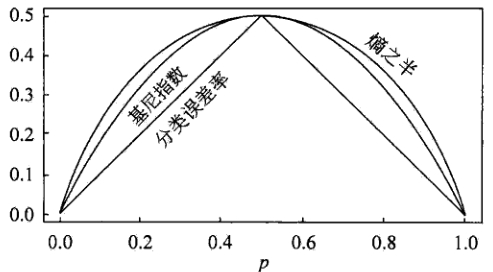ÕģČõĖŁ，µ©¬ÕØÉµĀćĶĪ©ńż║µ”éńÄć

p

p

Õłåń▒╗µĀæńÜäÕē¬µ×Ø
ķóäÕē¬µ×Ø
Õ«Üõ╣ēõĖĆõĖ¬ķ½śÕ║”，ÕĮōÕå│ńŁ¢µĀæĶŠŠÕł░Ķ»źķ½śÕ║”µŚČÕ░▒Õü£µŁóÕå│ńŁ¢µĀæńÜäńö¤ķĢ┐ĶŠŠÕł░µ¤ÉõĖ¬ĶŖéńé╣ńÜäÕ«×õŠŗÕģĘµ£ēńøĖÕÉīńÜäńē╣ÕŠüÕÉæķćÅ，ÕŹ│õĮ┐Ķ┐Öõ║øÕ«×õŠŗõĖŹÕ▒×õ║ÄÕÉīõĖĆń▒╗，õ╣¤ÕÅ»õ╗źÕü£µŁóÕå│ńŁ¢µĀæńÜäńö¤ķĢ┐ŃĆéĶ┐ÖõĖ¬µ¢╣µ│ĢÕ»╣õ║ÄÕżäńÉåµĢ░µŹ«ńÜäµĢ░µŹ«Õå▓ń¬üķŚ«ķóśµ»öĶŠāµ£ēµĢłŃĆéÕ«Üõ╣ēõĖĆõĖ¬ķśłÕĆ╝，ÕĮōĶŠŠÕł░µ¤ÉõĖ¬ĶŖéńé╣ńÜäÕ«×õŠŗõĖ¬µĢ░Õ░Åõ║ÄķśłÕĆ╝µŚČÕ░▒ÕÅ»õ╗źÕü£µŁóÕå│ńŁ¢µĀæńÜäńö¤ķĢ┐Õ«Üõ╣ēõĖĆõĖ¬ķśłÕĆ╝，ķĆÜĶ┐ćĶ«Īń«Śµ»Åµ¼Īµē®Õ╝ĀÕ»╣ń│╗ń╗¤µĆ¦ĶāĮńÜäÕó×ńøŖ，Õ╣Čµ»öĶŠāÕó×ńøŖÕĆ╝õĖÄĶ»źķśłÕĆ╝Õż¦Õ░ÅµØźÕå│Õ«Üµś»ÕÉ”Õü£µŁóÕå│ńŁ¢µĀæńÜäńö¤ķĢ┐ŃĆé
ÕÉÄÕē¬µ×Ø
ķöÖĶ»»ńÄćķÖŹõĮÄÕē¬µ×Ø（REP，Reduced-Error Pruning）
ķöÖĶ»»ńÄćķÖŹõĮÄÕē¬µ×Øµ¢╣µ│ĢĶĆāÕ░åµĀæõĖŖńÜäµ»ÅõĖ¬ĶŖéńé╣õĮ£õĖ║õ┐«Õē¬ńÜäÕĆÖķĆēÕ»╣Ķ▒Ī，ÕåŹÕå│Õ«Üµś»Õ»╣Ķ»źĶŖéńé╣Ķ┐øĶĪīÕē¬µ×Ø：
ÕłĀķÖżõ╗źµŁżń╗ōńé╣õĖ║µĀ╣ńÜäÕŁÉµĀæõĮ┐ÕģČµłÉõĖ║ÕÅČÕŁÉń╗ōńé╣ÕĮōõ┐«Õē¬ÕÉÄńÜäµĀæÕ»╣õ║Äķ¬īĶ»üķøåÕÉłńÜäµĆ¦ĶāĮõĖŹµ»öõ┐«Õē¬ÕēŹńÜäµĀæńÜäµĆ¦ĶāĮÕĘ«µŚČ，ÕłÖńĪ«Ķ«żÕłĀķÖżĶ»źń╗ōńé╣，ÕÉ”ÕłÖµüóÕżŹĶ»źĶŖéńé╣
ÕøĀõĖ║Ķ«Łń╗āķøåÕÉłńÜäĶ┐ćµŗ¤ÕÉł，õĮ┐ÕŠŚķ¬īĶ»üķøåÕÉłµĢ░µŹ«ĶāĮÕż¤Õ»╣ÕģČĶ┐øĶĪīõ┐«µŁŻ，ÕÅŹÕżŹĶ┐øĶĪīõĖŖķØóńÜäµōŹõĮ£，õ╗ÄÕ║ĢÕÉæõĖŖńÜäÕżäńÉåń╗ōńé╣，ÕłĀķÖżķéŻõ║øĶāĮÕż¤µÅÉķ½śķ¬īĶ»üķøåÕÉłńÜäń▓ŠÕ║”ńÜäń╗ōńé╣，ńø┤Õł░Ķ┐øõĖĆµŁźõ┐«Õē¬õ╝ÜķÖŹõĮÄķ¬īĶ»üķøåÕÉłńÜäń▓ŠÕ║”õĖ║µŁóŃĆé
µé▓Ķ¦éķöÖĶ»»Õē¬µ×Ø（PEP，Pesimistic-Error Pruning）

N

N

õĖ¬µĀĘµ£¼，ÕģČõĖŁµ£ē

E

E

õĖ¬ķöÖĶ»»，ķéŻõ╣łĶ»źÕÅČÕŁÉĶŖéńé╣ńÜäķöÖĶ»»ńÄćõĖ║

E

+

0.5

N

\frac{E + 0.5}{N}

ŃĆéĶ┐ÖõĖ¬

0.5

0.5

Õ░▒µś»µā®ńĮÜÕøĀÕŁÉ，ķéŻõ╣łõĖĆķóŚÕŁÉµĀæ，Õ«āµ£ē

L

L

õĖ¬ÕÅČÕŁÉĶŖéńé╣，ķéŻõ╣łĶ»źÕŁÉµĀæńÜäĶ»»ÕłżńÄćõ╝░Ķ«ĪõĖ║：

Ōłæ

E

i

+

0.5

ŌłŚ

L

Ōłæ

N

i

\frac{\sum E_i +0.5 * L}{\sum N_i}

E

E

õ╣¤ķ£ĆĶ”üÕŖĀõĖŖõĖĆõĖ¬µā®ńĮÜÕøĀÕŁÉ，ÕÅśµłÉ

E

+

0.5

E+0.5

，ķéŻõ╣łÕŁÉµĀæµś»ÕÉ”ÕÅ»õ╗źĶó½Õē¬µ×ØÕ░▒ÕÅ¢Õå│õ║ÄÕē¬µ×ØÕÉÄńÜäķöÖĶ»»

E

+

0.5

E+0.5

e

e

E

+

0.5

<

E

i

+

S

E

(

E

i

)

E+0.5 < E_i + SE(E_i)

ÕłÖÕ»╣

i

i

Ķ┐øĶĪīÕē¬µ×ØŃĆé
õ╗Żõ╗ĘÕżŹµØéÕ║”Õē¬µ×Ø（CCP，Cost-Complexity Pruning)
õ╗Żõ╗ĘÕżŹµØéÕ║”Õē¬µ×Øń«Śµ│ĢõĖ║ÕŁÉµĀæ

T

t

T_t

╬▒

╬▒

T

t

T_t

T

t

T_t

ÕćÅÕ░æńÜäÕÅČń╗ōńé╣µĢ░，

╬▒

╬▒

╬▒

=

R

(

t

)

ŌłÆ

R

(

T

t

)

ŌłŻ

N

t

ŌłŻ

ŌłÆ

1

\alpha = \frac{R(t) - R(T_t)}{|N_t| - 1}

ÕģČõĖŁ，

ŌłŻ

N

t

ŌłŻ

|N_t|

µś»ÕŁÉµĀæ

T

t

T_t

õĖŁńÜäÕÅČĶŖéńé╣µĢ░，

R

(

t

)

=

r

(

t

)

ŌłŚ

p

(

t

)

R(t) = r(t) * p(t)

õĖ║ń╗ōńé╣

t

t

ńÜäķöÖĶ»»õ╗Żõ╗Ę，

r

(

t

)

r(t)

õĖ║ń╗ōńé╣

t

t

ńÜäķöÖÕłåµĀĘµ£¼ńÄć，

p

(

t

)

p(t)

õĖ║ĶÉĮÕģźń╗ōńé╣

t

t

ńÜäµĀĘµ£¼ÕŹĀµēĆµ£ēµĀĘµ£¼ńÜäµ»öõŠŗ，

R

(

T

t

)

=

Ōłæ

R

(

i

)

R(T_t) = \sum R(i)

µś»ÕŁÉµĀæ

T

t

T_t

ķöÖĶ»»õ╗Żõ╗Ę，

i

i

õĖ║ÕŁÉµĀæ

T

t

T_t

ńÜäÕÅČĶŖéńé╣ŃĆé

T

T

ńÜäµ»ÅõĖ¬ķØ×ÕÅČń╗ōńé╣Ķ«Īń«Ś

╬▒

╬▒

ÕĆ╝，ÕŠ¬ńÄ»Õē¬µÄēÕģĘµ£ēµ£ĆÕ░Å

╬▒

╬▒

ÕĆ╝ńÜäÕŁÉµĀæ，ńø┤Õł░Õē®õĖŗµĀ╣ĶŖéńé╣，ÕŠŚÕł░õĖĆń│╗ÕłŚńÜäÕē¬µ×ØµĀæ

{

T

0

,

T

ŌĆś

1

,

T

2

,

Ōŗ»
ŌĆē

,

T

m

}

\{ T_0, T_1, T_2, \cdots, T_m \}

，ÕģČõĖŁ

T

0

T_0

T

m

T_m

õĖ║µĀ╣ń╗ōńé╣，

T

i

+

1

T_{i +1}

õĖ║Õ»╣

T

i

T_i

Ķ┐øĶĪīÕē¬µ×ØńÜäń╗ōµ×£õ╗ÄÕŁÉµĀæÕ║ÅÕłŚõĖŁ，µĀ╣µŹ«ń£¤Õ«×ńÜäĶ»»ÕĘ«õ╝░Ķ«ĪķĆēµŗ®µ£ĆõĮ│Õå│ńŁ¢µĀæ
REPPEPCCPÕē¬µ×Øµ¢╣Õ╝ÅĶć¬Õ║ĢÕÉæõĖŖĶć¬ķĪČÕÉæõĖŗĶć¬Õ║ĢÕÉæõĖŖĶ«Īń«ŚÕżŹµØéÕ║”

O

(

n

)

O(n)

O

(

n

)

O(n)

O

(

n

2

)

O(n^2)

Õø×ÕĮÆµĀæ
Õ░åķóäµĄŗÕÅśķćÅń®║ķŚ┤（

X

1

,

X

2

,

X

3

,

Ōŗ»
ŌĆē

,

X

p

X_1, X_2, X_3, \cdots, X_p

）ńÜäÕÅ»ĶāĮÕÅ¢ÕĆ╝µ×äµłÉńÜäķøåÕÉłÕłåÕē▓µłÉ

J

J

õĖ¬õ║ÆõĖŹķćŹÕÅĀńÜäÕī║Õ¤¤

{

R

1

,

R

2

,

R

3

,

Ōŗ»
ŌĆē

,

R

J

}

\{ R_1, R_2, R_3, \cdots, R_J\}

Õ»╣ĶÉĮÕģźÕī║Õ¤¤

R

j

R_j

ńÜäµ»ÅõĖ¬Ķ¦éµĄŗÕĆ╝õĮ£ÕÉīµĀĘńÜäķóäµĄŗ，ķóäµĄŗÕĆ╝ńŁēõ║Ä

R

j

R_j

õĖŖĶ«Łń╗āķøåńÜäÕÉäõĖ¬µĀĘµ£¼ÕÅ¢ÕĆ╝ńÜäń«Śµ£»Õ╣│ÕØćµĢ░ŃĆé

R

1

R_1

ÕÆī

R

2

R_2

，

R

1

R_1

õĖŁĶ«Łń╗āķøåńÜäÕÉäõĖ¬µĀĘµ£¼ÕÅ¢ÕĆ╝ńÜäń«Śµ£»Õ╣│ÕØćµĢ░õĖ║10，

R

2

R_2

õĖŁĶ«Łń╗āķøåńÜäÕÉäõĖ¬µĀĘµ£¼ÕÅ¢ÕĆ╝ńÜäń«Śµ£»Õ╣│ÕØćµĢ░õĖ║20ŃĆéÕłÖÕ»╣ń╗ÖÕ«ÜńÜäĶ¦éµĄŗÕĆ╝

X

=

x

X = x

，Ķŗź

x

Ōłł

R

1

x \in R_1

，ń╗ÖÕć║ńÜäķóäµĄŗÕĆ╝õĖ║10，Ķŗź

x

Ōłł

R

2

x \in R_2

，ÕłÖķóäµĄŗÕĆ╝õĖ║20ŃĆé

{

R

1

,

R

2

,

R

3

,

Ōŗ»
ŌĆē

,

R

J

}

\{ R_1, R_2, R_3, \cdots, R_J\}

R

S

S

µ£ĆÕ░ÅńÜäń¤®ÕĮóÕī║Õ¤¤

{

R

1

,

R

2

,

R

3

,

Ōŗ»
ŌĆē

,

R

J

}

\{ R_1, R_2, R_3, \cdots, R_J\}

ŃĆé

R

S

S

ńÜäÕ«Üõ╣ēõĖ║：

R

S

S

=

Ōłæ

j

=

1

J

Ōłæ

i

Ōłł

R

j

(

y

i

ŌłÆ

y

^

R

j

)

2

RSS = \sum^J_{j=1} \sum_{i \in R_j}(y_i - \hat{y}_{R_j})^2

ÕģČõĖŁ，

y

^

R

j

\hat{y}_{R_j}

µś»ń¼¼

j

j

õĖ¬ń¤®ÕĮóÕī║Õ¤¤õĖŁĶ«Łń╗āķøåõĖŁÕÉäõĖ¬µĀĘµ£¼ÕÅ¢ÕĆ╝ńÜäń«Śµ£»Õ╣│ÕØćµĢ░ŃĆéõĮåµś»，Ķ”üµā│ĶĆāĶÖæÕ░åńē╣ÕŠüń®║ķŚ┤ÕłÆÕłåõĖ║

J

J

X

j

X_j

ÕÆīÕłåÕē▓ńé╣

s

s

，Õ░åķóäµĄŗÕÅśķćÅń®║ķŚ┤ÕłåõĖ║õĖżõĖ¬Õī║Õ¤¤

{

X

ŌłŻ

X

j

<

s

}

\{ X|X_j <s \}

ÕÆī

{

X

ŌłŻ

X

j

Ōēź

s

}

\{ X|X_j \geq s \}

，õĮ┐

R

S

S

Õ░ĮÕÅ»ĶāĮÕ£░ÕćÅÕ░ÅŃĆéõ╣¤Õ░▒µś»Ķ»┤，ĶĆāĶÖæµēĆµ£ēķóäµĄŗÕÅśķćÅ

X

1

,

X

2

,

X

3

,

Ōŗ»
ŌĆē

,

X

p

X_1, X_2, X_3, \cdots, X_p

ÕÆīõĖÄµ»ÅõĖ¬ķóäµĄŗÕÅśķćÅÕ»╣Õ║öńÜä

s

s

ńÜäÕÅ¢ÕĆ╝，ńäČÕÉÄķĆēµŗ®ķóäµĄŗÕÅśķćÅÕÆīÕłåÕē▓ńé╣，õĮ┐µ×äķĆĀÕć║ńÜäµĀæÕģĘµ£ēµ£ĆÕ░ÅńÜä

R

S

S

ŃĆéµø┤Ķ»”ń╗åÕ£░，Õ»╣

j

j

ÕÆī

s

s

，Õ«Üõ╣ēõĖĆÕ»╣ÕŹŖÕ╣│ķØó：

R

1

(

j

,

s

)

=

{

X

ŌłŻ

X

j

<

s

}

ÕÆī

R

2

(

j

,

s

)

=

{

X

ŌłŻ

X

j

Ōēź

s

}

R_1(j, s) = \{ X|X_j <s \} \quad \text{ÕÆī} \quad R_2(j, s) = \{ X|X_j \geq s \}

Õ»╗µēŠ

j

j

ÕÆī

s

s

，õĮ┐ÕŠŚõĖŗÕ╝Åµ£ĆÕ░Å：

Ōłæ

x

i

Ōłł

R

1

(

j

,

s

)

(

y

i

ŌłÆ

y

^

R

1

)

2

+

Ōłæ

x

i

Ōłł

R

2

(

j

,

s

)

(

y

i

ŌłÆ

y

^

R

2

)

2

\sum_{x_i \in R_1(j, s)}(y_i - \hat{y}_{R_1})^2 + \sum_{x_i \in R_2(j, s)}(y_i - \hat{y}_{R_2})^2

ķćŹÕżŹõĖŖĶ┐░µŁźķ¬ż，Õ»╗µēŠń╗¦ń╗ŁÕłåÕē▓µĢ░µŹ«ķøåńÜäµ£Ćõ╝śķóäµĄŗÕÅśķćÅÕÆīµ£Ćõ╝śÕłåÕē▓ńé╣，õĮ┐ķÜÅõ╣ŗõ║¦ńö¤ńÜäÕī║Õ¤¤õĖŁńÜä

R

S

S

ĶŠŠÕł░µ£ĆÕ░ÅŃĆéµŁżµŚČĶó½ÕłåÕē▓ńÜäõĖŹÕåŹµś»µĢ┤õĖ¬ķóäµĄŗÕÅśķćÅń®║ķŚ┤，ĶĆīµś»õ╣ŗÕēŹńĪ«Õ«ÜńÜäõĖżõĖ¬Õī║Õ¤¤õ╣ŗõĖĆŃĆéÕ”éµŁżõĖĆµØźÕ░▒ĶāĮÕŠŚÕł░3õĖ¬Õī║Õ¤¤ŃĆéµÄźńØĆĶ┐øõĖĆµŁźÕłåÕē▓3õĖ¬Õī║Õ¤¤õ╣ŗõĖĆõ╗źµ£ĆÕ░ÅÕī¢

R

S

S

Õī║Õ¤¤

{

R

1

,

R

2

,

R

3

,

Ōŗ»
ŌĆē

,

R

J

}

\{ R_1, R_2, R_3, \cdots, R_J\}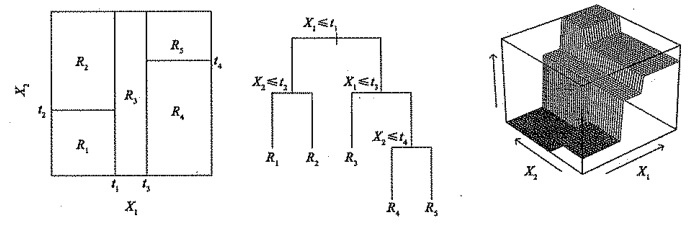Õø×ÕĮÆµĀæńÜäÕē¬µ×Ø

{

R

1

,

R

2

,

R

3

,

Ōŗ»
ŌĆē

,

R

J

}

\{ R_1, R_2, R_3, \cdots, R_J\}

ńÜäõĖ¬µĢ░µø┤Õ░æ）ńÜäµĀæõ╝Üµ£ēµø┤Õ░ÅńÜäµ¢╣ÕĘ«ÕÆīµø┤ÕźĮńÜäÕÅ»Ķ¦ŻķćŖµĆ¦（õ╗źÕó×ÕŖĀÕŠ«Õ░ÅÕüÅÕĘ«õĖ║õ╗Żõ╗Ę）ŃĆéķÆłÕ»╣õĖŖĶ┐░ķŚ«ķóś，õĖĆń¦ŹÕÅ»ĶāĮńÜäĶ¦ŻÕå│ÕŖ×µ│Ģµś»：õ╗ģÕĮōÕłåĶŻéõĮ┐µ«ŗÕĘ«Õ╣│µ¢╣ÕÆī

R

S

S

R

S

S

õ╝ÜÕż¦Õ╣ģÕćÅÕ░ÅŃĆé
ÕøĀµŁż，µø┤ÕźĮńÜäńŁ¢ńĢźµś»ńö¤µłÉõĖĆµŻĄÕŠłÕż¦ńÜäµĀæ

T

0

T_0

╬▒

\alpha

µĀćĶ«░ńÜäõĖĆń│╗ÕłŚÕŁÉµĀæŃĆéµ»ÅõĖĆõĖ¬

╬▒

\alpha

ńÜäÕÅ¢ÕĆ╝Õ»╣Õ║öõĖĆµŻĄÕŁÉµĀæ

T

Ōłł

T

0

T \in T_0

，ÕĮō

╬▒

\alpha

õĖĆÕ«ÜµŚČ，ÕģČÕ»╣Õ║öńÜäÕŁÉµĀæõĮ┐õĖŗÕ╝Åµ£ĆÕ░Å：

Ōłæ

m

=

1

ŌłŻ

T

ŌłŻ

Ōłæ

x

i

Ōłł

R

m

(

y

i

ŌłÆ

y

^

R

m

)

2

+

╬▒

ŌłŻ

T

ŌłŻ

\sum_{m=1}^{|T|} \sum_{x_i \in R_m}(y_i - \hat{y}_{R_m})^2 + \alpha |T|

Ķ┐ÖķćīńÜä

ŌłŻ

T

ŌłŻ

|T|

T

T

ńÜäń╗ōńé╣µĢ░，

R

m

R_m

µś»ń¼¼

m

m

õĖ¬ń╗łń½»ń╗ōńé╣Õ»╣Õ║öńÜäń¤®ÕĮó（ķóäµĄŗÕÉæķćÅń®║ķŚ┤ńÜäõĖĆõĖ¬ÕŁÉķøå），

y

^

R

m

\hat{y}_{R_m}

µś»õĖÄ

R

m

R_m

Õ»╣Õ║öńÜäķóäµĄŗÕĆ╝，õ╣¤Õ░▒µś»

R

m

R_m

õĖŁĶ«Łń╗āķøåńÜäÕ╣│ÕØćÕĆ╝ŃĆéĶ░āµĢ┤ń│╗µĢ░

╬▒

\alpha

╬▒

=

0

\alpha = 0

µŚČ，ÕŁÉµĀæ

T

T

ńŁēõ║ÄÕÄ¤µĀæ

T

0

T_0

，ÕøĀõĖ║µŁżµŚČõĖŖÕ╝ÅÕÅ¬ĶĪĪķćÅõ║åĶ«Łń╗āĶ»»ÕĘ«ŃĆéĶĆīÕĮō

╬▒

\alpha

Õó×Õż¦µŚČ，ń╗łń½»ń╗ōńé╣µĢ░ÕżÜńÜäµĀæÕ░åõĖ║Õ«āńÜäÕżŹµØéõ╗śÕć║õ╗Żõ╗Ę，µēĆõ╗źõĮ┐õĖŖÕ╝ÅÕÅ¢Õł░µ£ĆÕ░ÅÕĆ╝ńÜäÕŁÉµĀæõ╝ÜÕÅśÕŠŚµø┤Õ░ÅŃĆé
ÕĮō

╬▒

\alpha

╬▒

\alpha

╬▒

\alpha

╬▒

\alpha

K

K

µŖśõ║żÕÅēķ¬īĶ»×ķĆēµŗ®

╬▒

\alpha

ŃĆéÕģĘõĮōÕüÜµ│Ģµś»Õ░åĶ«Łń╗āķøåÕłåõĖ║

K

K

µŖśŃĆéÕ»╣µēĆµ£ē

k

=

1

,

2

,

3

,

Ōŗ»
ŌĆē

,

K

k=1, 2, 3, \cdots, K

，Õ»╣Ķ«Łń╗āķøåõĖŖµēĆµ£ēõĖŹÕ▒×õ║Äń¼¼

k

k

µŖśńÜäµĢ░µŹ«ķćŹÕżŹń¼¼(1)µŁź~ń¼¼(2)µŁźÕŠŚÕł░õĖÄ

╬▒

\alpha

k

k

µŖśõĖŖńÜäÕØćµ¢╣ķóäµĄŗĶ»»ÕĘ«ŃĆé 4.µ»ÅõĖ¬

╬▒

\alpha

õ╝Üµ£ēńøĖÕ║öńÜä

K

K

õĖ¬ÕØćµ¢╣ķóäµĄŗĶ»»ÕĘ«，Õ»╣Ķ┐Ö

K

K

õĖ¬ÕĆ╝µ▒éÕ╣│ÕØć，ķĆēÕć║õĮ┐Õ╣│ÕØćĶ»»ÕĘ«µ£ĆÕ░ÅńÜä

╬▒

\alpha

ŃĆé 5.µēŠÕć║ķĆēÕ«ÜńÜä

╬▒

\alpha

Õå│ńŁ¢µĀæńÜäÕ¤║ńĪĆÕŹ│µś»õĖŖµ¢ćµēĆĶ┐░ńÜäÕłåń▒╗Õå│ńŁ¢µĀæõĖÄÕø×ÕĮÆÕå│ńŁ¢µĀæ，ÕģČķóäµĄŗÕćåńĪ«µĆ¦õĖĆĶł¼µ»öÕø×ÕĮÆÕÆīÕłåń▒╗µ¢╣µ│ĢÕ╝▒，õĮåÕÅ»õ╗źķĆÜĶ┐ćńö©ķøåµłÉÕŁ”õ╣Āµ¢╣µ│Ģń╗äÕÉłÕż¦ķćÅÕå│ńŁ¢µĀæ，µśŠĶæŚµÅÉÕŹćµĀæńÜäķóäµĄŗµĢłµ×£，Ķ┐Öõ║øµ¢╣µ│ĢµłæÕ░åõ╝ÜÕ£©µÄźõĖŗµØźńÜäµ¢ćń½ĀõĖŁń╗¦ń╗ŁķśÉĶ┐░，µ¼óĶ┐ÄÕģ│µ│©ÕŁ”õ╣ĀĶ«©Ķ«║！
Õ▒ĢÕ╝ĆÕģ©µ¢ćµ£║ÕÖ©ÕŁ”õ╣Ā cartÕłåń▒╗Õø×ÕĮÆµĀæ Õø×ÕĮÆµĀæ
ŌĆāŌĆāÕå│ńŁ¢µĀæ（decision tree）µś»õĖĆń¦ŹÕ¤║µ£¼ńÜäÕłåń▒╗õĖÄÕø×ÕĮÆµ¢╣µ│Ģ，µ£¼µ¢ćõĖ╗Ķ”üĶ«©Ķ«║ńö©õ║ÄÕłåń▒╗ńÜäÕå│ńŁ¢µĀæŃĆéÕå│ńŁ¢µĀæÕŁ”õ╣ĀķĆÜÕĖĖÕīģµŗ¼õĖēõĖ¬µŁźķ¬ż：ńē╣ÕŠüķĆēµŗ®，Õå│ńŁ¢µĀæńÜäńö¤µłÉÕÆīÕå│ńŁ¢µĀæńÜäõ┐«Õē¬ŃĆéĶĆīķÜÅµ£║µŻ«µ×ŚÕłÖµś»ńö▒ÕżÜõĖ¬Õå│ńŁ¢µĀæµēĆµ×äµłÉńÜäõĖĆń¦ŹÕłåń▒╗ÕÖ©，µø┤ÕćåńĪ«ńÜäĶ»┤，ķÜÅµ£║µŻ«µ×Śµś»ńö▒ÕżÜõĖ¬Õ╝▒Õłåń▒╗ÕÖ©ń╗äÕÉłÕĮóµłÉńÜäÕ╝║Õłåń▒╗ÕÖ©ŃĆé

ńø«ÕĮĢ
õĖĆŃĆüõ┐Īµü»Ķ«║ń¤źĶ»å1.õ┐Īµü»ńåĄńÜäµ”éÕ┐Ą2.µØĪõ╗ČńåĄ3.ńøĖÕ»╣ńåĄ4.õ║Æõ┐Īµü»5.ÕćĀõĖ¬ķćÅõ╣ŗķŚ┤ńÜäÕģ│ń│╗
õ║īŃĆüÕå│ńŁ¢µĀæ1.Õ╝ĢÕģź2.Õå│ńŁ¢µĀæńÜäńö¤µłÉń«Śµ│Ģ1.õ┐Īµü»Õó×ńøŖõĖÄID32.õ┐Īµü»Õó×ńøŖńÄćõĖÄC4.53.Giniń│╗µĢ░õĖÄCART
3.Õå│ńŁ¢µĀæńÜäĶ»äõ╗Ę4.Õå│ńŁ¢µĀæńÜäĶ┐ćµŗ¤ÕÉł1.Õē¬µ×Ø

õĖēŃĆüķÜÅµ£║µŻ«µ×Ś1.Bootstrap2.baggingńŁ¢ńĢź3.out of bag estimate（ÕīģÕż¢õ╝░Ķ«Ī）4.µĀĘµ£¼ķÜÅµ£║õĖÄńē╣ÕŠüķÜÅµ£║

õĖĆŃĆüõ┐Īµü»Ķ«║ń¤źĶ»å
1.õ┐Īµü»ńåĄńÜäµ”éÕ┐Ą
ŌĆāŌĆāĶ«Šń”╗µĢŻÕ×ŗķÜÅµ£║ÕÅśķćÅXńÜäÕÅ¢ÕĆ╝µ£ē

x

1

,

x

2

,

x

3

,

.

.

.

,

x

n

x_{1},x_{2},x_{3},...,x_{n}

，ÕģČÕÅæńö¤µ”éńÄćÕłåÕł½õĖ║

p

1

,

p

2

,

p

3

,

.

.

.

,

p

n

p_{1},p_{2},p_{3},...,p_{n}

，ķéŻõ╣łÕ░▒ÕÅ»õ╗źÕ«Üõ╣ēõ┐Īµü»ńåĄõĖ║：ŌĆāŌĆāõĖĆĶł¼Õ»╣µĢ░ńÜäÕ║ĢµĢ░µś»2，ÕĮōńäČõ╣¤ÕÅ»õ╗źµŹóµłÉe，ÕĮōÕ»╣µĢ░Õ║ĢµĢ░õĖ║2µŚČ，õ┐Īµü»ńåĄńÜäÕŹĢõĮŹõĖ║µ»öńē╣，õ┐Īµü»ńåĄõ╣¤ń¦░ķ”ÖÕå£ńåĄŃĆéÕĮōÕ»╣µĢ░õĖŹõĖ║2ĶĆīµś»ÕģČõ╗¢Õż¦õ║Ä2ńÜäµĢ┤µĢ░rµŚČ，µłæõ╗¼ń¦░õ┐Īµü»ńåĄõĖ║r-Ķ┐øÕłČńåĄ，Ķ«░õĖ║

H

r

(

X

)

H_{r}(X)

，Õ«āõĖÄõ┐Īµü»ńåĄĶĮ¼µŹóÕģ¼Õ╝ÅõĖ║：ŌĆāŌĆāõ┐Īµü»ńåĄńö©õ╗źµÅÅĶ┐░õ┐Īµ║ÉńÜäõĖŹńĪ«Õ«ÜÕ║”， µ”éńÄćĶČŖÕż¦，ÕÅ»ĶāĮµĆ¦ĶČŖÕż¦，õĮåµś»õ┐Īµü»ķćÅĶČŖÕ░Å，õĖŹńĪ«Õ«ÜµĆ¦ĶČŖÕ░Å，ńåĄĶČŖÕ░ÅŃĆé
2.µØĪõ╗ČńåĄ
ŌĆāŌĆāĶ«ŠķÜÅµ£║ÕÅśķćÅ(X,Y)ÕģĘµ£ēĶüöÕÉłµ”éńÄćÕłåÕĖā：ŌĆāŌĆāµØĪõ╗ČńåĄ

H

(

Y

ŌłŻ

X

)

H(Y|X)

ĶĪ©ńż║ Õ£©ÕĘ▓ń¤źķÜÅµ£║ÕÅśķćÅXńÜäµØĪõ╗ČõĖŗķÜÅµ£║ÕÅśķćÅYńÜäõĖŹńĪ«Õ«ÜµĆ¦ŃĆé ŌĆāŌĆā ÕÅ»õ╗źĶ┐ÖµĀĘńÉåĶ¦Ż： ŌĆāŌĆā (X,Y)ÕÅæńö¤µēĆÕīģÕÉ½ńÜäńåĄ，ÕćÅÕÄ╗XÕŹĢńŗ¼ÕÅæńö¤ńÜäńåĄ，Õ░▒µś»Õ£©XÕÅæńö¤ńÜäÕēŹµÅÉõĖŗ，YÕÅæńö¤µ¢░ÕĖ”µØźńÜäńåĄŃĆé ŌĆāŌĆā µēĆõ╗źµØĪõ╗ČńåĄµ£ēÕ”éõĖŗÕģ¼Õ╝ÅµłÉń½ŗ：ŌĆāŌĆā µÄ©Õ»╝Õ”éõĖŗ：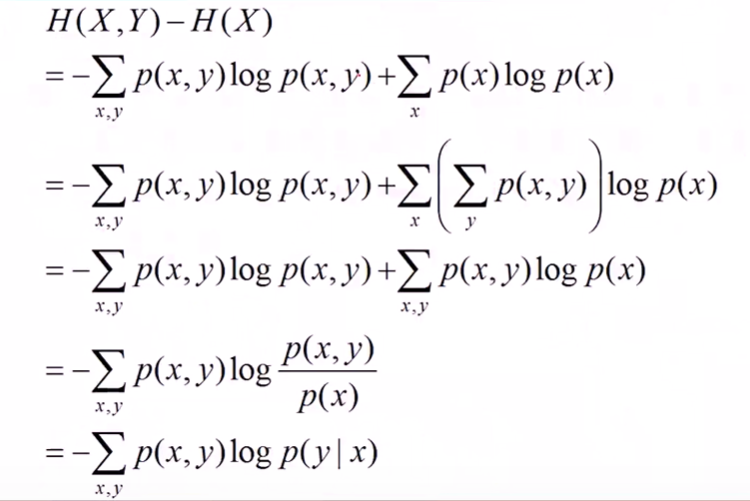3.ńøĖÕ»╣ńåĄ
ŌĆāŌĆā ńøĖÕ»╣ńåĄ，ÕÅłń¦░õ║ÆńåĄ，õ║żÕÅēńåĄ，ķē┤Õł½õ┐Īµü»，KullbackńåĄ，Kullback-LeibleµĢŻÕ║”ńŁēŃĆéÕ«Üõ╣ēÕ”éõĖŗ： Ķ«Šp(x)，q(x)µś»ķÜÅµ£║ÕÅśķćÅXõĖŁÕÅ¢ÕĆ╝ńÜäõĖżõĖ¬µ”éńÄćÕłåÕĖā，ÕłÖpÕ»╣qńÜäńøĖÕ»╣ńåĄõĖ║：ŌĆāŌĆāÕ£©õ┐Īµü»ńÉåĶ«║õĖŁ，ńøĖÕ»╣ńåĄńŁēõ╗Ęõ║ÄõĖżõĖ¬ÕłåÕĖāńÜäõ┐Īµü»ńåĄ（Shannon entropy）ńÜäÕĘ«ÕĆ╝ŃĆé ÕŹ│：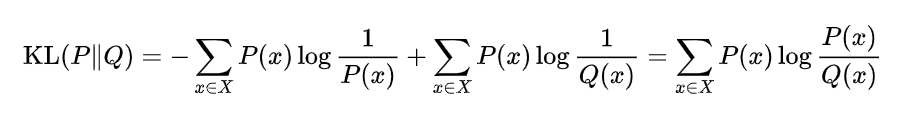4.õ║Æõ┐Īµü»
ŌĆāŌĆāõĖżõĖ¬ķÜÅµ£║ÕÅśķćÅXÕÆīYńÜäõ║Æõ┐Īµü»，Õ«Üõ╣ēõĖ║X，YńÜäĶüöÕÉłÕłåÕĖāÕÆīńŗ¼ń½ŗÕłåÕĖāõ╣śń¦»ńÜäńøĖÕ»╣ńåĄŃĆéÕŹ│：ŌĆāŌĆāµēĆõ╗źµĀ╣µŹ«KLµĢŻÕ║”õ╣¤Õ░▒µś»ńøĖÕ»╣ńåĄńÜäÕ«Üõ╣ē，ÕÅ»õ╗źµÄ©Õć║õ║Æõ┐Īµü»ńÜäĶĪ©ĶŠŠÕ╝ÅÕ”éõĖŗ：ŌĆāŌĆāń╗¦ń╗ŁµÄ©Õ»╝Õ”éõĖŗ：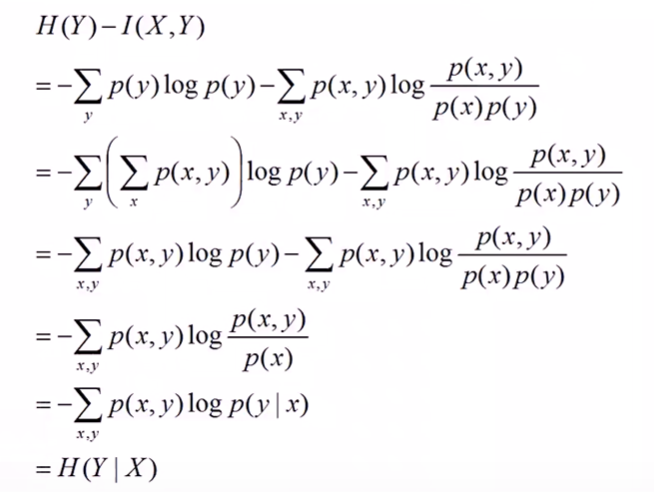ŌĆāŌĆāµēĆõ╗źµ£ĆÕÉÄµ£ē：5.ÕćĀõĖ¬ķćÅõ╣ŗķŚ┤ńÜäÕģ│ń│╗
ŌĆāŌĆāń╗ōÕÉłõĖŖĶ┐░µØĪõ╗ČńåĄńÜäõĖżõĖ¬ĶĪ©ĶŠŠÕ╝Å，ÕÅ»õ╗źĶ┐øõĖĆµŁźµÄ©Õć║：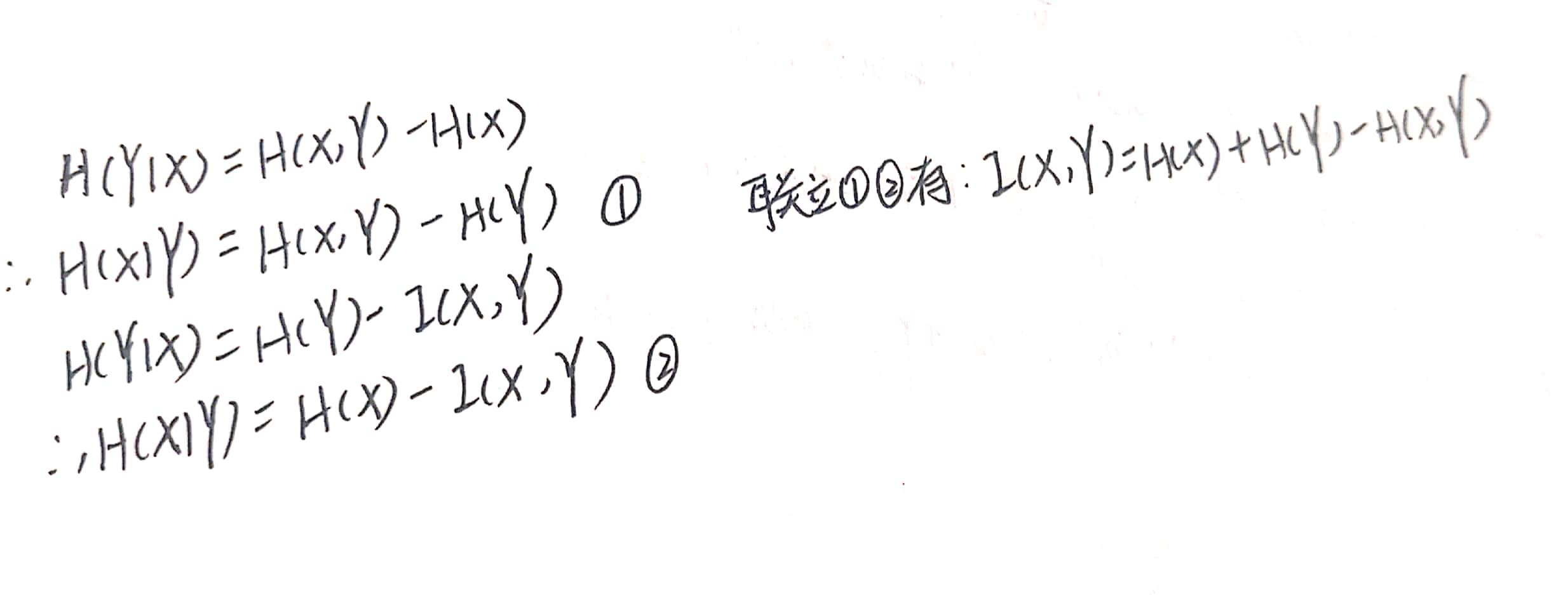ŌĆāŌĆāÕĮōńäČµłæõ╗¼õ╣¤ÕÅ»õ╗źµĀ╣µŹ«ńåĄńÜäÕ«Üõ╣ēµØźńø┤µÄźµÄ©Õć║õĖŖķØóĶ┐ÖõĖ¬õ║Æõ┐Īµü»ńÜäÕģ¼Õ╝Å：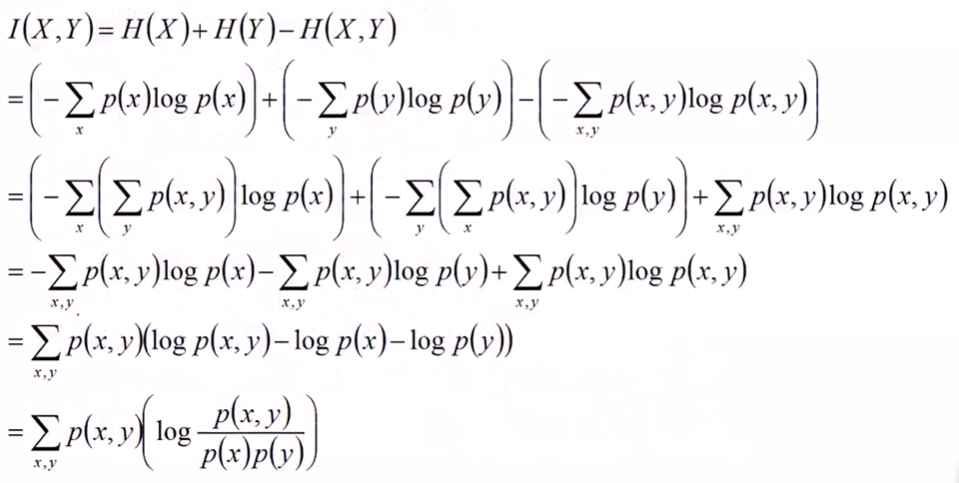ŌĆāŌĆāÕÉīµŚČµłæõ╗¼õ╣¤ÕÅ»õ╗źÕŠŚÕł░õĖżõĖ¬õĖŹńŁēÕ╝Å：ŌĆāŌĆāõĖŖķØóĶ┐ÖõĖ¬õĖŹńŁēÕ╝ÅÕæŖĶ»ēµłæõ╗¼，Õ»╣õ║ÄõĖĆõĖ¬õĖÄXńøĖÕģ│ńÜäķÜÅµ£║ÕÅśķćÅY，ÕÅ¬Ķ”üµłæõ╗¼ÕŠŚń¤źõ║åõĖĆńé╣Õģ│õ║ÄYńÜäõ┐Īµü»，ķéŻõ╣łXńÜäõĖŹńĪ«Õ«ÜÕ║”Õ░▒õ╝ÜÕćÅÕ░ÅŃĆé ŌĆāŌĆāµ£ĆÕÉÄ，ÕĆ¤ÕŖ®Õ╝║Õż¦ńÜäķ¤”µü®ÕøŠµØźĶ«░õĮÅĶ┐Öõ║øÕģ│ń│╗：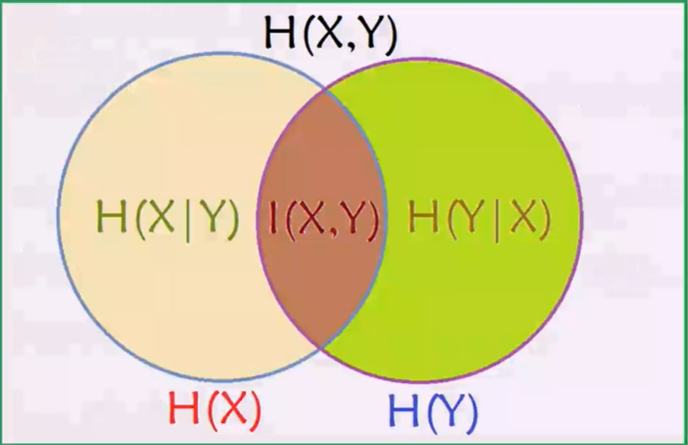õ║īŃĆüÕå│ńŁ¢µĀæ
1.Õ╝ĢÕģź
ŌĆāŌĆāÕå│ńŁ¢µĀæ，ķĪŠÕÉŹµĆØõ╣ē，Õ░▒µś»ÕĖ«µłæõ╗¼ÕüÜÕć║Õå│ńŁ¢ńÜäµĀæŃĆéńÄ░Õ«×ńö¤µ┤╗õĖŁµłæõ╗¼ÕŠĆÕŠĆõ╝ÜķüćÕł░ÕÉäń¦ŹÕÉäµĀĘńÜäµŖēµŗ®，µŖŖµłæõ╗¼ńÜäÕå│ńŁ¢Ķ┐ćń©ŗµĢ┤ńÉåõĖĆõĖŗ，Õ░▒ÕÅ»õ╗źÕÅæńÄ░，Ķ»źĶ┐ćń©ŗÕ«×ķÖģõĖŖÕ░▒µś»õĖĆõĖ¬µĀæńÜäµ©ĪÕ×ŗŃĆéµ»öÕ”éńøĖõ║▓ńÜäµŚČÕĆÖ：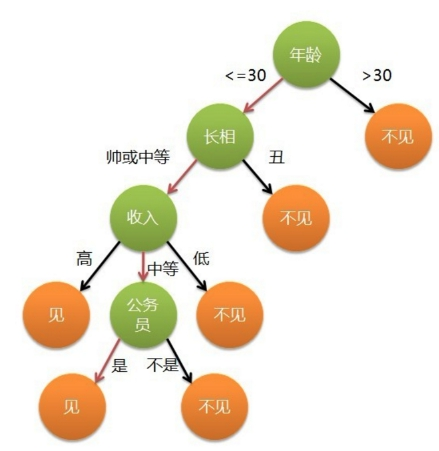ŌĆāŌĆāµłæõ╗¼ÕÅ»õ╗źĶ«żõĖ║Õ╣┤ķŠä，ķĢ┐ńøĖ，µöČÕģźµś»õĖĆõĖ¬õ║║ńÜäõĖēõĖ¬ńē╣ÕŠü，µ»Åµ¼Īµłæõ╗¼ÕüÜÕć║µŖēµŗ®ķāĮµś»Õ¤║õ║ÄĶ┐ÖõĖēõĖ¬ńē╣ÕŠüµØźµŖŖõĖĆõĖ¬ĶŖéńé╣ÕłåµłÉÕźĮÕćĀõĖ¬µ¢░ńÜäĶŖéńé╣ŃĆé ŌĆāŌĆāõĖĆķóŚÕ«īµĢ┤ńÜäÕå│ńŁ¢µĀæÕīģÕÉ½õ╗źõĖŗõĖēõĖ¬ķā©Õłå：
ŌĆāŌĆā µĀæõĖŁµ»ÅõĖ¬Õåģķā©ĶŖéńé╣ĶĪ©ńż║Õ£©õĖĆõĖ¬Õ▒×µĆ¦ńē╣ÕŠüõĖŖńÜäµĄŗĶ»Ģ，µ»ÅõĖ¬Õłåµö»õ╗ŻĶĪ©õĖĆõĖ¬µĄŗĶ»ĢĶŠōÕć║，µ»ÅõĖ¬ÕÅČĶŖéńé╣ĶĪ©ńż║õĖĆń¦Źń▒╗Õł½ŃĆé ń╗ÖÕ«ÜõĖĆõĖ¬Õå│ńŁ¢µĀæńÜäÕ«×õŠŗ：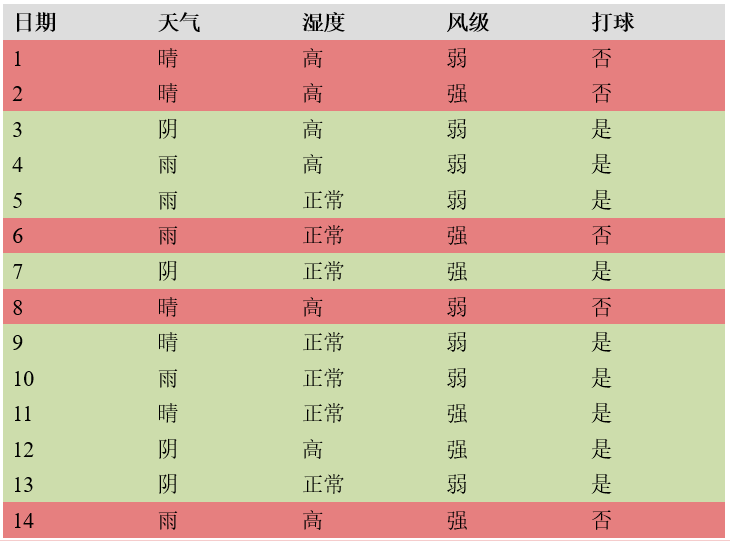ŌĆāŌĆā µ×äķĆĀÕå│ńŁ¢µĀæÕ”éõĖŗ：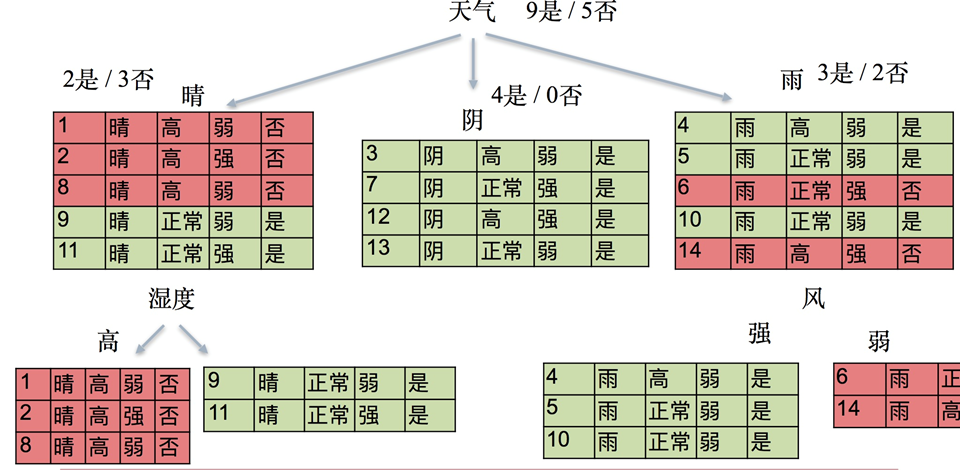1.ń¼¼õĖĆÕ▒éµĀ╣ĶŖéńé╣ Ķó½ÕłåµłÉ14õ╗Į，9µś»/5ÕÉ”，µĆ╗õĮōńÜäõ┐Īµü»ńåĄõĖ║： H0= -[(5/14)log5/14+(9/14)log9/14]=0.9403.
2.ń¼¼õ║īÕ▒é µÖ┤：Ķó½ÕłåõĖ║5õ╗Į，2µś»/3ÕÉ”，Õ«āńÜäõ┐Īµü»ńåĄõĖ║： H1=-[(2/5)log2/5+(3/5)log3/5]=0.9710. ķś┤：Ķó½ÕłåõĖ║4õ╗Į，4µś»/0ÕÉ”，Õ«āńÜäõ┐Īµü»ńåĄõĖ║： H2= -[log1]=0. ķø©：Ķó½ÕłåõĖ║5õ╗Į，3µś»/2ÕÉ”，Õ«āńÜäõ┐Īµü»ńåĄõĖ║： H3=-[(2/5)log2/5+(3/5)log3/5]=0.9710. ŌĆāŌĆā µłæõ╗¼Ķ¦äÕ«Ü，ÕüćĶ«Šµłæõ╗¼ķĆēÕÅ¢Õż®µ░öõĖ║Õłåń▒╗õŠØµŹ«，µŖŖÕ«āõĮ£õĖ║µĀ╣ĶŖéńé╣，ķéŻõ╣łń¼¼õ║īÕ▒éńÜäÕŖĀµØāõ┐Īµü»ńåĄ，õ╣¤Õ░▒µś»

H

ŌĆ▓

=

5

/

14

H

1

+

4

/

14

H

2

+

5

/

14

H

3

H^{'}=5/14H1+4/14H2+5/14H3

Ķ”üµ»öH0Õ░Å，õ╣¤Õ░▒µś»ķÜÅńØĆÕå│ńŁ¢ńÜäĶ┐øĶĪī，ÕģČõĖŹńĪ«Õ«ÜÕ║”Ķ”üÕćÅÕ░ÅµēŹĶĪī，Ķ”üõĖŹńäČµłæõ╗¼Ķ┐śÕå│ńŁ¢Õ╣▓õ╗Ćõ╣łŃĆéŃĆéŃĆéÕå│ńŁ¢Ķé»Õ«Üµś»õĖĆõĖ¬ńö▒õĖŹńĪ«Õ«ÜÕł░ńĪ«Õ«ÜńŖČµĆüńÜäĶĮ¼ÕÅśŃĆé ń«ŚÕć║

H

ŌĆ▓

=

0.6936

<

0.9403

H^{'}=0.6936<0.9403

，ń¼”ÕÉłĶ”üµ▒éŃĆé ŌĆāŌĆā õ║ŗÕ«×õĖŖ，ķÜÅńØĆÕłåń▒╗ńÜäĶ┐øĶĪī，ĶČŖµØźĶČŖÕżÜńÜäõ┐Īµü»Ķó½ń¤źķüō，ķéŻõ╣łµĆ╗õĮōńÜäńåĄĶé»Õ«ÜÕ«×µāĀõĖŗķÖŹńÜäŃĆé ŌĆāŌĆāÕÉīµĀĘ，Õ»╣õ║ÄµÖ┤Ķ┐ÖõĖ¬ĶŖéńé╣，õ╗¢ńÜäõĖżõĖ¬ÕÅČÕŁÉń╗ōńé╣ńÜäńåĄķāĮµś»0，Õł░õ║åÕÅČÕŁÉń╗ōńé╣õ╣ŗÕÉÄ，ńåĄÕ░▒ÕÅśõĖ║0õ║å，Õ░▒ÕŠŚÕł░õ║åÕå│ńŁ¢ń╗ōµ×£ŃĆé ŌĆāŌĆā ÕøĀµŁż，Õå│ńŁ¢µĀæķććńö©ńÜäµś»Ķć¬ķĪČÕÉæõĖŗńÜäķĆÆÕĮÆµ¢╣µ│Ģ，ÕģČÕ¤║µ£¼µĆØµā│µś»õ╗źõ┐Īµü»ńåĄõĖ║Õ║”ķćÅµ×äķĆĀõĖĆµŻĄńåĄÕĆ╝õĖŗķÖŹµ£ĆÕ┐½ńÜäµĀæ，Õł░ÕÅČÕŁÉĶŖéńé╣ÕżäńÜäńåĄÕĆ╝õĖ║0，µŁżµŚČµ»ÅõĖ¬ÕÅČÕŁÉĶŖéńé╣õĖŁńÜäÕ«×õŠŗķāĮÕ▒×õ║ÄÕÉīõĖĆń▒╗ŃĆé Ķć│õ║ÄµĆÄõ╣łÕ«Üõ╣ēõĖŗķÖŹµ£ĆÕ┐½，õĖŗķØóµÄźńØĆĶ«▓ŃĆé
2.Õå│ńŁ¢µĀæńÜäńö¤µłÉń«Śµ│Ģ
1.õ┐Īµü»Õó×ńøŖõĖÄID3
ŌĆāŌĆāÕå│ńŁ¢µĀæõĖŁõ┐Īµü»Õó×ńøŖÕ«Üõ╣ēÕ”éõĖŗ： ŌĆāŌĆāń╗ÖÕ«ÜõĖĆõĖ¬µĀĘµ£¼ķøåD，ÕłÆÕłåÕēŹµĀĘµ£¼ķøåÕÉłDńÜäńåĄµś»õĖĆÕ«ÜńÜä ，ńö©H0ĶĪ©ńż║； õĮ┐ńö©µ¤ÉõĖ¬ńē╣ÕŠüAÕłÆÕłåµĢ░µŹ«ķøåD，Ķ«Īń«ŚÕłÆÕłåÕÉÄńÜäµĢ░µŹ«ÕŁÉķøåńÜäńåĄ，ńö©H1ĶĪ©ńż║，ÕłÖ： ŌĆāŌĆāõ┐Īµü»Õó×ńøŖ=H0-H1，õ╣¤ÕÅ»õ╗źĶĪ©ńż║õĖ║：ŌĆāŌĆāµ»öÕ”éõĖŖķØóÕ«×õŠŗõĖŁµłæķĆēµŗ®Õż®µ░öõĮ£õĖ║µĀ╣ĶŖéńé╣，Õ░åµĀ╣ĶŖéńé╣õĖĆÕłåõĖ║õĖē，Ķ«Šf1ĶĪ©ńż║Õż®µ░ö，ÕłÖµ£ē:

g

(

D

,

f

1

)

=

H

0

ŌłÆ

H

ŌĆ▓

=

0.9403

ŌłÆ

0.6936

=

0.2467.

g(D,f1)=H0-H^{'}=0.9403-0.6936=0.2467.

2.õ┐Īµü»Õó×ńøŖńÄćõĖÄC4.5
ŌĆāŌĆāõĖ║õ║åĶ¦ŻÕå│õ┐Īµü»Õó×ńøŖńÜäÕ▒ĆķÖÉ，Õ╝ĢÕģźõ║åõ┐Īµü»Õó×ńøŖńÄćńÜäµ”éÕ┐ĄŃĆéÕłåµö»Ķ┐ćÕżÜÕ«╣µśōÕ»╝Ķć┤Ķ┐ćµŗ¤ÕÉł，ķĆĀµłÉõĖŹńÉåµā│ńÜäÕÉÄµ×£ŃĆéÕüćĶ«ŠÕÄ¤µØźńÜäńåĄõĖ║0.9，ķĆēµŗ®f1ńē╣ÕŠüÕłÆÕłåÕÉÄµĢ┤õĮōńåĄÕÅśµłÉõ║å0.1，õ╣¤Õ░▒µś»õ┐Īµü»Õó×ńøŖõĖ║0.8，ĶĆīķĆēµŗ®f2ÕłÆÕłåÕÉÄ，ńåĄÕÅśõĖ║0.3，õ╣¤Õ░▒µś»õ┐Īµü»Õó×ńøŖõĖ║0.6ŃĆéµłæõ╗¼õĖŹµā│ķĆēµŗ®f1，ÕøĀõĖ║Õ«āĶ«®Õå│ńŁ¢µĀæÕłåµö»Õż¬ÕżÜõ║å，ķéŻõ╣łÕ░▒ÕÅ»õ╗źÕ«Üõ╣ēÕå│ńŁ¢µīćµĀć=õ┐Īµü»Õó×ńøŖ/ńē╣ÕŠüµ£¼Ķ║½ńÜäńåĄŃĆéf1ÕłÆÕłåÕÉÄÕłåµö»µø┤ÕżÜ，õ╣¤Õ░▒µś»ńē╣ÕŠüf1µ£¼Ķ║½ńÜäńåĄµ»öf2µø┤Õż¦，Õż¦ńÜäµĢ░ķÖżõ╗źõĖĆõĖ¬Õż¦ńÜäµĢ░，ÕłÜÕźĮÕÅ»õ╗źõĖŁÕÆīõĖĆõĖŗŃĆé ŌĆāŌĆāÕŹ│：ŌĆāŌĆāĶ┐śµś»õ╗źĶ┐ÖÕ╝ĀÕøŠõĖ║õŠŗÕŁÉń«ŚõĖĆõĖŗ：ńÄ░Õ£©µłæõ╗¼Ķ”üķĆēµŗ®Ķ░üÕüÜµĀ╣ĶŖéńé╣ŃĆéµ£¬ÕłÆÕłåÕēŹ：（ÕēŹķØóķāĮń«ŚĶ┐ćõĖĆķüŹõ║å） H0= 0.9403. ķĆēµŗ®Õż®µ░öõĮ£õĖ║µĀ╣ĶŖéńé╣，ÕłÖµ£ē： µÖ┤：Ķó½ÕłåõĖ║5õ╗Į，2µś»/3ÕÉ”，Õ«āńÜäõ┐Īµü»ńåĄõĖ║： H1=0.9710. ķś┤：Ķó½ÕłåõĖ║4õ╗Į，4µś»/0ÕÉ”，Õ«āńÜäõ┐Īµü»ńåĄõĖ║： H2= -[log1]=0. ķø©：Ķó½ÕłåõĖ║5õ╗Į，3µś»/2ÕÉ”，Õ«āńÜäõ┐Īµü»ńåĄõĖ║： H3=0.9710. ÕłÆÕłåÕÉÄµĆ╗õĮōńÜäõ┐Īµü»ńåĄõĖ║：

H

ŌĆ▓

=

5

/

14

H

1

+

4

/

14

H

2

+

5

/

14

H

3

=

0.6936

H^{'}=5/14H1+4/14H2+5/14H3=0.6936

H

0

=

ŌłÆ

[

(

5

/

14

)

l

o

g

5

/

14

+

(

4

/

14

)

l

o

g

4

/

14

+

(

5

/

14

)

l

o

g

5

/

14

]

=

0.9403

=

1.5774

H0= -[(5/14)log5/14+(4/14)log4/14+(5/14)log5/14]=0.9403=1.5774

. ķéŻõ╣łķĆēµŗ®Õż®µ░öõĮ£õĖ║Õłåń▒╗õŠØµŹ«µŚČ，Ķ»äõ╗ĘµīćµĀć=0.2467/1.5774=0.1566.
3.Giniń│╗µĢ░õĖÄCART
ŌĆāŌĆāÕ«Üõ╣ē：Õ¤║Õ░╝µīćµĢ░（Õ¤║Õ░╝õĖŹń║»Õ║”）：ĶĪ©ńż║Õ£©µĀĘµ£¼ķøåÕÉłõĖŁõĖĆõĖ¬ķÜÅµ£║ķĆēõĖŁńÜäµĀĘµ£¼Ķó½ÕłåķöÖńÜäµ”éńÄćŃĆé ŌĆāŌĆāÕ«Üõ╣ēÕ╝Å：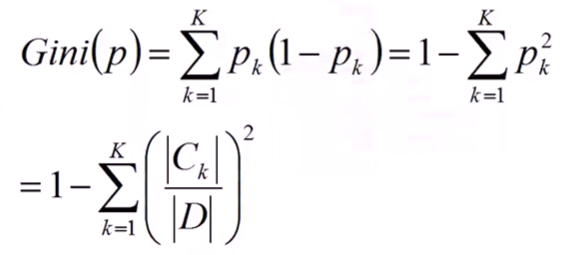õĖĆõ║øÕÅéµĢ░ńÜäĶ»┤µśÄ：
ŌĆāŌĆāÕģ│õ║ÄÕ¤║Õ░╝ń│╗µĢ░ńÜäńÉåĶ¦Ż，ńĮæõĖŖµ£ēõĖĆń¦ŹĶ»┤µ│Ģµ»öĶŠāķĆÜõ┐ŚµśōµćéŃĆéńÄ░Ķ¦ŻķćŖÕ”éõĖŗ： ŌĆāŌĆāµłæõ╗¼ń¤źķüōõ┐Īµü»ńåĄńÜäÕ«Üõ╣ēÕ╝ÅõĖ║：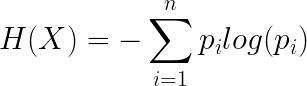ŌĆāŌĆāķéŻõ╣łÕ¤║Õ░╝ń│╗µĢ░Õ«×ķÖģõĖŖÕ░▒µś»ńö©

1

ŌłÆ

p

i

1-p_{i}

µØźõ╗Żµø┐õ║å

ŌłÆ

l

o

g

(

p

i

)

-log(p_{i})

，ńö╗Õć║õ║īĶĆģÕøŠÕāÅ：ŌĆāŌĆāÕøĀõĖ║µ”éńÄćµś»Õ▒×õ║Ä0Õł░1õ╣ŗķŚ┤，µēĆõ╗źµłæõ╗¼ÕÅ¬ń£ŗ01Õī║ķŚ┤õĖŖńÜäÕøŠÕāÅ：Õ¤║Õ░╝ń│╗µĢ░Õ»╣õ║Äõ┐Īµü»ńåĄĶĆīĶ©Ć，Õ░▒µś»Õ£©01Õī║ķŚ┤ÕåģĶ┐æõ╝╝ńÜäńö©Õłćń║┐µØźõ╗Żµø┐õ║åÕ»╣µĢ░ÕćĮµĢ░ŃĆéÕøĀµŁż，µŚóńäČõ┐Īµü»ńåĄÕÅ»õ╗źĶĪ©Ķ┐░õĖŹńĪ«Õ«ÜÕ║”，ķéŻõ╣łÕ¤║Õ░╝ń│╗µĢ░Ķć¬ńäČõ╣¤ÕÅ»õ╗ź，ÕÅ¬õĖŹĶ┐ćÕŁśÕ£©õĖĆõ║øĶ»»ÕĘ«ŃĆé
3.Õå│ńŁ¢µĀæńÜäĶ»äõ╗Ę
ŌĆāŌĆāÕüćÕ«ÜµĀĘµ£¼µĆ╗ń▒╗Õł½õĖ║K,ĶĆīÕ»╣õ║ÄÕå│ńŁ¢µĀæńÜäµ¤ÉÕÅČĶŖéńé╣，ÕüćÕ«ÜĶ»źÕÅČÕŁÉĶŖéńé╣ÕīģÕÉ½ńÜäµĀĘµ£¼µĢ░ńø«õĖ║n，ÕģČõĖŁń¼¼kń▒╗ńÜäµĀĘµ£¼µĢ░ńø«õĖ║

n

k

n_{k}

，k=1,2,ŌĆ”,KŃĆé Ķŗźµ¤Éń▒╗µĀĘµ£¼ńÜä

n

j

n_{j}

l

o

g

K

logK

ŃĆé ŌĆāŌĆāķéŻõ╣łµłæõ╗¼Õ»╣µēĆµ£ēÕÅČÕŁÉń╗ōńé╣ńÜäńåĄµ▒éÕÆī，Ķ»źÕĆ╝ĶČŖÕ░ÅĶ»┤µśÄĶČŖń▓ŠńĪ«ŃĆé ĶĆīÕÅłńö▒õ║ÄÕÉäõĖ¬ÕÅČÕŁÉń╗ōńé╣ÕīģÕÉ½ńÜäµĀĘµ£¼µĢ░ńø«õĖŹÕÉī，µēĆõ╗źµłæõ╗¼ķććńö©ÕŖĀµØāńåĄÕÆīŃĆé ŌĆāŌĆāĶ»äõ╗ĘÕćĮµĢ░Õ«Üõ╣ēÕ”éõĖŗ：ŌĆāŌĆāÕģČõĖŁ

N

t

N_{t}

4.Õå│ńŁ¢µĀæńÜäĶ┐ćµŗ¤ÕÉł
1.Õē¬µ×Ø

T

╬▒

T_{╬▒}

õĖēŃĆüķÜÅµ£║µŻ«µ×Ś
ŌĆāŌĆāķÜÅµ£║µŻ«µ×Śõ╣¤µś»õĖ║õ║åĶ¦ŻÕå│Õå│ńŁ¢µĀæńÜäĶ┐ćµŗ¤ÕÉłķŚ«ķóśŃĆé
1.Bootstrap
2.baggingńŁ¢ńĢź
3.out of bag estimate（ÕīģÕż¢õ╝░Ķ«Ī）

(

1

ŌłÆ

1

/

N

)

N

(1-1/N)^N

4.µĀĘµ£¼ķÜÅµ£║õĖÄńē╣ÕŠüķÜÅµ£║
ŌĆāŌĆāÕ£©µłæõ╗¼õĮ┐ńö©Bootstrapńö¤µłÉmõĖ¬µĀĘµ£¼ķøåµŚČ，µ»ÅõĖĆõĖ¬µĀĘµ£¼ķøåńÜäµĀĘµ£¼µĢ░ńø«õĖŹõĖĆÕ«ÜĶ”üńŁēõ║ÄÕÄ¤Õ¦ŗµĀĘµ£¼ķøåńÜäµĀĘµ£¼µĢ░ńø«，µ»öÕ”éµłæõ╗¼ÕÅ»õ╗źńö¤µłÉõĖĆõĖ¬ÕÉ½µ£ē0.75NõĖ¬µĀĘµ£¼ńÜäµĀĘµ£¼ķøå，µŁżÕżä0.75Õ░▒ń¦░õĖ║ķććµĀĘńÄćŃĆé ŌĆāŌĆāÕÉīµĀĘ，µłæõ╗¼Õ£©Õł®ńö©0.75NõĖ¬µĀĘµ£¼ńö¤µłÉÕå│ńŁ¢µĀæµŚČ，ÕüćĶ«Šµłæõ╗¼ķććńö©ID3ń«Śµ│Ģ，ńö¤µłÉń╗ōńé╣µŚČõ╗źõ┐Īµü»Õó×ńøŖõĮ£õĖ║Õłżµ¢ŁõŠØµŹ«ŃĆéµłæõ╗¼ńÜäÕģĘõĮōÕüÜµ│Ģµś»µŖŖµ»ÅõĖĆõĖ¬ńē╣ÕŠüķāĮµŗ┐µØźĶ»ĢõĖĆĶ»Ģ，µ£Ćń╗łõ┐Īµü»Õó×ńøŖµ£ĆÕż¦ńÜäńē╣ÕŠüÕ░▒µś»µłæõ╗¼Ķ”üķĆēńÜäńē╣ÕŠüŃĆéõĮåµś»，µłæõ╗¼Õ£©ķĆēµŗ®ńē╣ÕŠüńÜäĶ┐ćń©ŗõĖŁ，õ╣¤ÕÅ»õ╗źÕÅ¬ķĆēµŗ®õĖĆķā©Õłåńē╣ÕŠü，µ»öÕ”é20õĖ¬ķćīķØóµłæÕÅ¬ķĆēµŗ®16õĖ¬ńē╣ÕŠüŃĆé ŌĆāŌĆāķéŻÕÅ»ĶāĮµ£ēńÜäõ║║Õ░▒Ķ”üķŚ«õ║å，ÕüćĶ«ŠõĮĀµ▓ĪķĆēńÜä4õĖ¬ńē╣ÕŠüķćīķØóÕłÜÕźĮµ£ēõĖĆõĖ¬µś»µ£ĆÕźĮńÜäÕæó？Ķ┐Öń¦ŹµāģÕåĄµś»Õ«īÕģ©ÕÅ»ĶāĮÕć║ńÄ░ńÜä，õĮåµś»µłæõ╗¼Õ£©õĖŗõĖĆµ¼ĪńÜäÕłåÕÅēĶ┐ćń©ŗõĖŁ，Ķ»źńē╣ÕŠüµś»µ£ēÕÅ»ĶāĮĶó½ķćŹµ¢░µŹĪÕø×µØźńÜä，ÕÅ”Õż¢Õł½ńÜäÕå│ńŁ¢µĀæÕĮōõĖŁõ╣¤ÕÅ»ĶāĮõ╝ÜÕć║ńÄ░ķéŻõ║øÕ£©ÕÅ”õĖĆķóŚÕå│ńŁ¢µĀæõĖŁµ▓Īµ£ēńö©Õł░ńÜäńē╣ÕŠüŃĆé ŌĆāŌĆāķÜÅµ£║µŻ«µ×ŚńÜäÕ«Üõ╣ēÕ░▒Õć║µØźõ║å，Õł®ńö©baggingńŁ¢ńĢźńö¤µłÉõĖĆńŠżÕå│ńŁ¢µĀæńÜäĶ┐ćń©ŗõĖŁ，Õ”éµ×£µłæõ╗¼ÕÅłµ╗ĪĶČ│õ║åµĀĘµ£¼ķÜÅµ£║ÕÆīńē╣ÕŠüķÜÅµ£║，ķéŻõ╣łµ×äÕ╗║ÕźĮńÜäĶ┐ÖõĖĆµē╣Õå│ńŁ¢µĀæ，µłæõ╗¼Õ░▒ń¦░õĖ║ķÜÅµ£║µŻ«µ×Ś(Random Forest)ŃĆé ŌĆāŌĆāÕ«×ķÖģõĖŖ，µłæõ╗¼õ╣¤ÕÅ»õ╗źõĮ┐ńö©SVM，ķĆ╗ĶŠæÕø×ÕĮÆńŁēõĮ£õĖ║Õłåń▒╗ÕÖ©，Ķ┐Öõ║øÕłåń▒╗ÕÖ©ń╗äµłÉńÜäµĆ╗Õłåń▒╗ÕÖ©，µłæõ╗¼õ╣Āµā»õĖŖõŠØµŚ¦ń¦░õĖ║ķÜÅµ£║µŻ«µ×ŚŃĆé
Õ▒ĢÕ╝ĆÕģ©µ¢ćÕ¤║Õ░╝ń│╗µĢ░ µ£║ÕÖ©ÕŁ”õ╣Ā õ┐Īµü»ńåĄ
• ## Õå│ńŁ¢µĀæÕÅŖÕå│ńŁ¢µĀæńö¤µłÉõĖÄÕē¬µ×Ø

õĖćµ¼ĪķśģĶ»╗ ÕżÜõ║║ńé╣ĶĄ× 2018-07-24 16:37:01
1. Õå│ńŁ¢µĀæÕŁ”õ╣Ā 2. µ£Ćõ╝śÕłÆÕłåÕ▒×µĆ¦ńÜäķĆēµŗ® 2.1 õ┐Īµü»Õó×ńøŖ - ID3 2.1.1 õ╗Ćõ╣łµś»õ┐Īµü»Õó×ńøŖ 2.1.2 ID3 µĀæõĖŁµ£Ćõ╝śÕłÆÕłåÕ▒×µĆ¦Ķ«Īń«ŚõĖŠõŠŗ 2.2 õ┐Īµü»Õó×ńøŖńÄć - C4.5 2.3 Õ¤║Õ░╝µīćµĢ░ - CART 3. Õå│ńŁ¢µĀæÕē¬µ×Ø 3.1 Õå│ńŁ¢µĀæńÜäµŹ¤Õż▒...


µ¢ćń½Āńø«ÕĮĢ
1. Õå│ńŁ¢µĀæÕŁ”õ╣Ā2. µ£Ćõ╝śÕłÆÕłåÕ▒×µĆ¦ńÜäķĆēµŗ®2.1 õ┐Īµü»Õó×ńøŖ - ID32.1.1 õ╗Ćõ╣łµś»õ┐Īµü»Õó×ńøŖ2.1.2 ID3 µĀæõĖŁµ£Ćõ╝śÕłÆÕłåÕ▒×µĆ¦Ķ«Īń«ŚõĖŠõŠŗ
2.2 õ┐Īµü»Õó×ńøŖńÄć - C4.52.3 Õ¤║Õ░╝µīćµĢ░ - CART
3. Õå│ńŁ¢µĀæÕē¬µ×Ø3.1 Õå│ńŁ¢µĀæńÜäµŹ¤Õż▒ÕćĮµĢ░3.2 Õ”éõĮĢĶ┐øĶĪīÕå│ńŁ¢µĀæÕē¬µ×Ø3.2.1 ķóäÕē¬µ×Ø3.2.2 ÕÉÄÕē¬µ×Ø3.3.3 õĖżń¦ŹÕē¬µ×ØńŁ¢ńĢźÕ»╣µ»ö

ÕÅéĶĆā

(ĶĮ¼Ķć¬õĖ¬õ║║ÕŹÜÕ«ó)
Õå│ńŁ¢µĀæ (Decision tree) µś»õĖĆń¦ŹÕ¤║µ£¼ńÜäÕłåń▒╗õĖÄÕø×ÕĮÆµ¢╣µ│ĢŃĆéÕ«āµś»õĖĆõĖ¬µĀæÕĮóń╗ōµ×ä，Õ»╣õ║ÄµīćÕ«Üńē╣ÕŠüń®║ķŚ┤õĖŖńÜäµĢ░µŹ«ńé╣µØźĶ»┤，µĆ╗ĶāĮķĪ║ńØĆÕå│ńŁ¢µĀæńÜäµĀ╣ĶŖéńé╣õĖĆµŁźµŁźÕłåķģŹÕł░ÕŁÉĶŖéńé╣µ£Ćń╗łÕł░ĶŠŠÕÅČĶŖéńé╣，ĶĆīÕÅČĶŖéńé╣ĶĪ©ńż║õ║åĶ»źµĢ░µŹ«ńé╣µēĆÕ▒×ńÜäÕłåń▒╗ŃĆéÕ£©µ»ÅõĖĆµ¼ĪÕłåķģŹÕł░ÕŁÉĶŖéńé╣ńÜäĶ┐ćń©ŗõĖŁÕÅ»õ╗źń£ŗõĮ£µś»Õ»╣µĢ░µŹ«ńé╣õĖŁńē╣µ£ēńÜäńē╣ÕŠüÕ▒×µĆ¦ÕĆ╝Ķ┐øĶĪīńÜä if-then Õłżµ¢ŁŃĆé
Õå│ńŁ¢µĀæÕÅ»õ╗źĶ«żõĖ║µś» if-then Ķ¦äÕłÖńÜäķøåÕÉł，õ╣¤ÕÅ»õ╗źĶ«żõĖ║µŚČÕ«Üõ╣ēÕ£©ńē╣ÕŠüń®║ķŚ┤õĖÄń▒╗ń®║ķŚ┤õĖŖńÜäµØĪõ╗Čµ”éńÄćÕłåÕĖāŃĆéÕģČõĖ╗Ķ”üõ╝śńé╣µś»µ©ĪÕ×ŗÕģĘµ£ēÕÅ»Ķ»╗µĆ¦，Õłåń▒╗ķĆ¤Õ║”Õ┐½ŃĆéÕ”éõĮĢÕŠŚÕł░Ķ»źÕå│ńŁ¢µĀæÕÅ½ÕüÜÕå│ńŁ¢µĀæÕŁ”õ╣Ā，Õå│ńŁ¢µĀæÕŁ”õ╣ĀµŚČ，Õł®ńö©Ķ«Łń╗āµĢ░µŹ«，µĀ╣µŹ«µŹ¤Õż▒ÕćĮµĢ░µ£ĆÕ░ÅÕī¢ńÜäÕÄ¤ÕłÖÕ╗║ń½ŗÕå│ńŁ¢µĀæµ©ĪÕ×ŗŃĆéķóäµĄŗĶ»Ģ，Õ»╣µ¢░ńÜäµĢ░µŹ«，Õł®ńö©Õå│ńŁ¢µĀæµ©ĪÕ×ŗĶ┐øĶĪīÕłåń▒╗ŃĆé

1. Õå│ńŁ¢µĀæÕŁ”õ╣Ā
Õå│ńŁ¢µĀæÕŁ”õ╣ĀńÜäńø«ńÜäµś»õĖ║õ║åńö¤µłÉõĖĆķóŚµ│øÕī¢ĶāĮÕŖøÕ╝║，ÕŹ│ÕżäńÉåµ£¬Ķ¦üńż║õŠŗĶāĮÕŖøÕ╝║ńÜäÕå│ńŁ¢µĀæ，ÕģČÕ¤║µ£¼µĄüń©ŗķüĄÕŠ¬ń«ĆÕŹĢĶĆīńø┤Ķ¦éńÜä ÕłåĶĆīµ▓╗õ╣ŗ (Divide and Conquer) ńŁ¢ńĢź，Õ”éõĖŗÕøŠµēĆńż║：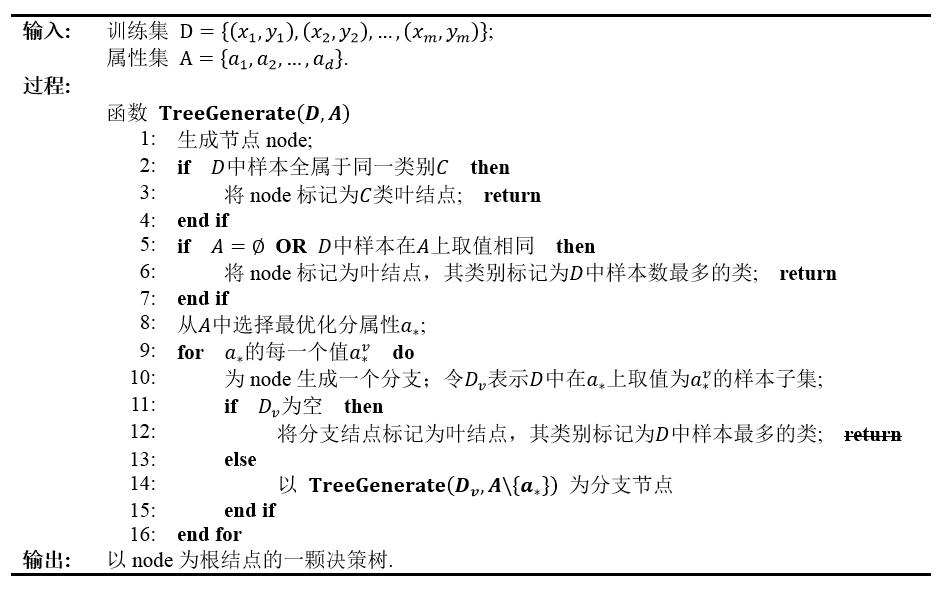(ĶĪī 3) ÕĮōÕēŹń╗ōńé╣ÕīģÕÉ½ńÜäµĀĘµ£¼Õģ©ķā©Õ▒×õ║ÄÕÉīõĖĆõĖ¬ń▒╗Õł½，µŚĀķ£ĆÕłÆÕłå；(ĶĪī 6) ÕĮōÕēŹÕ▒×µĆ¦ķøåõĖ║ń®║，µł¢µś»µēĆµ£ēµĀĘµ£¼Õ£©µēĆµ£ēÕ▒×µĆ¦õĖŖÕÅ¢ÕĆ╝ńøĖÕÉī，µŚĀµ│ĢÕłÆÕłåŃĆéÕ£©Ķ┐Öń¦ŹµāģÕåĄõĖŗ，µŖŖÕĮōÕēŹń╗ōńé╣µĀćĶ«░õĖ║ÕÅČń╗ōńé╣，Õ╣ČõĖöÕ░åÕģČń▒╗Õł½Ķ«ŠÕ«ÜõĖ║Ķ»źń╗ōńé╣µēĆÕÉ½µĀĘµ£¼µ£ĆÕżÜńÜäń▒╗Õł½；(ĶĪī 12) ÕĮōÕēŹń╗ōńé╣ÕīģÕÉ½ńÜäµĀĘµ£¼ķøåÕÆīõĖ║ń®║，õĖŹĶāĮÕłÆÕłå，µŖŖÕĮōÕēŹń╗ōńé╣µĀćĶ«░õĖ║ÕÅČń╗ōńé╣，õĮåµś»Õ░åÕģČń▒╗Õł½Ķ«ŠÕ«ÜõĖ║ÕģČńłČń╗ōńé╣µēĆÕÉ½µĀĘµ£¼µ£ĆÕżÜńÜäń▒╗Õł½，Õæ©Õ┐ŚÕŹÄĶĆüÕĖłńÜäŃĆŖµ£║ÕÖ©ÕŁ”õ╣ĀŃĆŗõĖŁÕ£©Ķ»źµØĪõ╗ČõĖŗµē¦ĶĪīõ║å return，õĮåµś»µīēńģ¦µłæńÜäńÉåĶ¦Żńö▒õ║ÄĶ┐ÖķćīÕżäõ║Ä for ÕŠ¬ńÄ»õĖŁ，ĶÖĮńäČÕ▒×µĆ¦õĖŁńÜäõĖĆõĖ¬ÕÅ¢ÕĆ╝µĀĘµ£¼ķøåÕÉłõĖ║ń®║，õĮåµś»ÕģČÕ«āÕÅ¢ÕĆ╝µāģÕåĄõĖŗĶ┐śµ£ēµ£ēÕÅ»ĶāĮµ£ēµĀĘµ£¼ķøåÕÉłńÜä，Õ”éµ×£Ķ┐Öķćīµē¦ĶĪīõ║å return，ķéŻõ╣łÕ░▒ĶĘ│Ķ┐ćõ║åÕģČÕ«āÕÅ¢ÕĆ╝Õłżµ¢ŁńÜäÕÅ»ĶāĮŃĆé
ÕÅ”Õż¢，ÕģČõĖŁń¼¼ 14 ĶĪī

A

\

{

a

ŌłŚ

}

A \backslash \lbrace a_\ast \rbrace

A

A

õĖŁÕÄ╗ķÖż

a

ŌłŚ

a_\ast

Õ▒×µĆ¦ŃĆé
2. µ£Ćõ╝śÕłÆÕłåÕ▒×µĆ¦ńÜäķĆēµŗ®
õ╗ÄķĆÆÕĮÆńö¤µłÉõ╝¬õ╗ŻńĀüÕøŠńż║õĖŁÕÅ»õ╗źń£ŗÕć║，(ĶĪī 8)ķĆēµŗ®µ£Ćõ╝śÕłÆÕłåÕ▒×µĆ¦

a

ŌłŚ

a_\ast

a

ŌłŚ

a_\ast

õ╣ŗÕÉÄ，ÕģČÕłåµö»ĶŖéńé╣µēĆÕīģÕÉ½ńÜäµĀĘµ£¼Õ░ĮÕÅ»ĶāĮÕ▒×õ║ÄÕÉīõĖĆń▒╗Õł½，ÕŹ│ń╗ōńé╣ńÜä ń║»Õ║” (Purity) ĶČŖµØźĶČŖķ½śŃĆé
µĀ╣µŹ«µ£Ćõ╝śÕ▒×µĆ¦

a

ŌłŚ

a_\ast

ķĆēµŗ®µ¢╣µ│ĢńÜäõĖŹÕÉī，Õå│ńŁ¢µĀæÕż¦Ķć┤ÕłåõĖ║õ║å ID3 [Quinlan, 1986]ŃĆüC4.5 [Quinlan, 1993]ŃĆüCART [Breiman et al., 1984]ŃĆé

4.1

4.1

õĖŁńÜäĶź┐ńō£µĢ░µŹ«Õ”éõĖŗ：
2.1 õ┐Īµü»Õó×ńøŖ - ID3
2.1.1 õ╗Ćõ╣łµś»õ┐Īµü»Õó×ńøŖ

4.2.1

4.2.1

Õ░ÅĶŖéõĖŁµēĆĶ┐░，ÕüćÕ«Üń”╗µĢŻÕ▒×µĆ¦

a

a

µ£ē

V

V

õĖ¬ÕÅ»ĶāĮńÜäÕÅ¢ÕĆ╝

{

a

1

,

a

2

,

.

.

.

,

a

V

}

\lbrace a_1,a_2,...,a_V \rbrace

a

a

µØźÕ»╣µĀĘµ£¼ķøå

D

D

Ķ┐øĶĪīÕłÆÕłå，õ╝Üõ║¦ńö¤

V

V

õĖ¬Õłåµö»ń╗ōńé╣，ÕģČõĖŁń¼¼

v

v

õĖ¬Õłåµö»ń╗ōńé╣ÕīģÕÉ½õ║å

D

D

a

a

õĖŖÕÅ¢ÕĆ╝õĖ║

a

v

a_v

ńÜäµĀĘµ£¼，Ķ«░õĖ║

D

v

D_v

，ÕģČÕ»╣Õ║öńÜäõ┐Īµü»ńåĄõĖ║：

H

(

D

v

)

=

ŌłÆ

Ōłæ

k

Ōłł

Y

p

(

k

ŌłŻ

v

)

log

ŌüĪ

p

(

k

ŌłŻ

v

)

=

ŌłÆ

Ōłæ

k

Ōłł

Y

ŌłŻ

D

v

k

ŌłŻ

ŌłŻ

D

v

ŌłŻ

log

ŌüĪ

ŌłŻ

D

v

k

ŌłŻ

ŌłŻ

D

v

ŌłŻ

H(D_v)=-\sum_{k \in \mathcal{Y}}p(k\mid v)\log p(k \mid v)=-\sum_{k \in \mathcal{Y}}\frac{|D_{vk}|}{|D_v|}\log \frac{|D_{vk}|}{|D_v|}

ÕģČõĖŁ

D

v

k

D_{vk}

D

v

D_v

õĖŁÕłåń▒╗õĖ║

k

k

ńÜäµĀĘµ£¼ŃĆéÕåŹĶĆāĶÖæÕł░õĖŹÕÉīńÜäÕłåµö»

v

v

ń╗ōńé╣µēĆÕīģÕÉ½ńÜäµĀĘµ£¼µĢ░õĖŹÕÉī，ń╗ÖÕłåµö»ń╗ōńé╣ĶĄŗõ║łµØāķćŹ

ŌłŻ

D

v

ŌłŻ

/

ŌłŻ

D

ŌłŻ

|D_v|/|D|

，õ║Äµś»ÕÅ»õ╗źĶ«Īń«ŚÕć║Õ▒×µĆ¦

a

a

Õ»╣µĀĘµ£¼ķøå

D

D

Ķ┐øĶĪīÕłÆÕłåµēĆĶÄĘÕŠŚńÜä õ┐Īµü»Õó×ńøŖ (Information gain)：

Gain

(

D

,

a

)

=

H

(

D

)

ŌłÆ

Ōłæ

v

=

1

V

ŌłŻ

D

v

ŌłŻ

ŌłŻ

D

ŌłŻ

H

(

ŌłŻ

D

v

ŌłŻ

)

=

H

(

D

)

ŌłÆ

H

(

D

,

a

)

=

I

(

D

,

a

)

\textrm{Gain}(D,a)=H(D)-\sum_{v=1}^V \frac{|D_v|}{|D|}H(|D_v|)=H(D)-H(D,a)=I(D,a)

ÕģČÕ«×，õ┐Īµü»Õó×ńøŖÕ░▒µś»Ķ«Łń╗āµĢ░µŹ«ķøå

D

D

a

a

µØźĶ┐øĶĪīÕłÆÕłåµēĆĶÄĘÕŠŚńÜäŌĆ£ń║»Õ║”µÅÉÕŹćŌĆØĶČŖÕż¦ŃĆéĶĆāĶÖæµ×üń½»µāģÕåĄ，ÕĮōÕ▒×µĆ¦

a

a

0

0

a

a

a

a

a

a

ÕĮōÕå│ńŁ¢µĀæõĖŁķĆēµŗ®µ£Ćõ╝śÕłÆÕłåÕ▒×µĆ¦（ĶĪī 8）µīēńģ¦õ┐Īµü»Õó×ńøŖµ£ĆÕż¦µØźĶ┐øĶĪīµŚČ，Õå│ńŁ¢µĀæÕ▒×õ║Ä ID3 Õå│ńŁ¢µĀæŃĆé
2.1.2 ID3 µĀæõĖŁµ£Ćõ╝śÕłÆÕłåÕ▒×µĆ¦Ķ«Īń«ŚõĖŠõŠŗ

2

2

(µś»ÕźĮńō£ŃĆüõĖŹµś»ÕźĮńō£)，ÕŹ│

Y

=

2

\mathcal{Y}=2

D

D

õĖŁµēĆµ£ēµĀĘõŠŗ，µŁŻõŠŗÕŹĀ

p

1

=

8

17

p_1=\frac{8}{17}

，ÕÅŹõŠŗÕŹĀ

p

2

=

9

17

p_2=\frac{9}{17}

ŃĆéµĀ╣ń╗ōńé╣ńÜäõ┐Īµü»ńåĄ（õĖŗķØóµłæõ╗¼ķāĮõ╗źµ»öńē╣õĖ║ÕŹĢõĮŹĶ«Īń«Ś）õĖ║：

H

(

D

)

=

ŌłÆ

Ōłæ

k

=

1

2

p

(

k

)

log

ŌüĪ

2

p

(

k

)

=

ŌłÆ

(

7

17

log

ŌüĪ

2

7

17

+

9

17

log

ŌüĪ

2

9

17

)

=

0.998

H(D)=-\sum_{k=1}^2p(k)\log_2 p(k)=-\left(\frac{7}{17}\log_2\frac{7}{17}+\frac{9}{17}\log_2\frac{9}{17}\right)=0.998

3

3

õĖ¬ÕÅ»ĶāĮÕÅ¢ÕĆ╝：{ķØÆń╗┐ŃĆüõ╣īķ╗æŃĆüµĄģńÖĮ}ŃĆéõ╗źĶ»źÕ▒×µĆ¦Õ»╣µĢ░µŹ«ķøåĶ┐øĶĪīÕłÆÕłå，ÕÅ»õ╗źÕŠŚÕł░

3

3

õĖ¬ÕŁÉķøå，ÕłåÕł½õĖ║：

D

1

D_1

(Ķē▓µ│Į=ķØÆń╗┐)ŃĆü

D

2

D_2

(Ķē▓µ│Į=õ╣īķ╗æ)ŃĆü

D

3

D_3

(Ķē▓µ│Į=µĄģńÖĮ)ŃĆé
Õ»╣ÕŁÉķøå

D

1

D_1

µØźĶ»┤，ÕīģÕÉ½õ║åń╝¢ÕÅĘõĖ║

{

1

,

4

,

6

,

10

,

13

,

17

}

\lbrace1,4,6,10,13,17\rbrace

ńÜä

6

6

õĖ¬µĀĘõŠŗ，ÕģČõĖŁµŁŻõŠŗõĖ║

{

1

,

4

,

6

}

\lbrace1,4,6\rbrace

，ÕŹĀ

p

1

=

3

6

p_1=\frac{3}{6}

；ÕÅŹõŠŗõĖ║

{

10

,

13

,

17

}

\lbrace10,13,17\rbrace

，ÕŹĀ

p

1

=

3

6

p_1=\frac{3}{6}

ŃĆéĶ«Īń«ŚÕģČńåĄõĖ║：

H

(

D

1

)

=

ŌłÆ

(

3

6

log

ŌüĪ

2

3

6

+

3

6

log

ŌüĪ

2

3

6

)

=

1.000

H(D_1)=-\left(\frac{3}{6}\log_2\frac{3}{6}+\frac{3}{6}\log_2\frac{3}{6}\right)=1.000

õŠØµ¼ĪÕÅ»õ╗źĶ«Īń«ŚÕÅ”Õż¢õĖżõĖ¬ÕŁÉķøåńÜäõ┐Īµü»ńåĄõĖ║：

H

(

D

2

)

=

0.918

H

(

D

3

)

=

0.722

\begin{aligned} H(D_2)&=0.918 \\ H(D_3)&=0.722 \end{aligned}

µ£Ćń╗łÕÅ»õ╗źĶ«Īń«ŚµĢ░µŹ«ķøå

D

D

Gain

(

D

,

Ķē▓

µ│Į

)

=

H

(

D

)

ŌłÆ

Ōłæ

v

=

1

3

p

(

v

)

H

(

D

v

)

=

0.998

ŌłÆ

(

6

17

├Ś

1.000

+

6

17

├Ś

0.918

+

5

17

├Ś

0.722

)

=

0.109

\begin{aligned} \textrm{Gain}(D,Ķē▓µ│Į) &=H(D)-\sum_{v=1}^3p(v)H(D_v) \\ &=0.998-\left(\frac{6}{17}\times1.000+\frac{6}{17}\times0.918+\frac{5}{17}\times0.722\right) \\ &=0.109 \end{aligned}

ķćŹÕżŹõĖŖĶ┐░ńÜäĶ«Īń«ŚµŁźķ¬ż，µłæõ╗¼ÕÅ»õ╗źĶ«Īń«ŚÕć║ÕģČõ╗¢Õ▒×µĆ¦ńÜäõ┐Īµü»Õó×ńøŖ：

Gain

(

D

,

µĀ╣

ĶÆé

)

=

0.143

;

Gain

(

D

,

µĢ▓

ÕŻ░

)

=

0.141

;

Gain

(

D

,

ń║╣

ńÉå

)

=

0.381

;

Gain

(

D

,

ĶäÉ

)

=

0.289

;

Gain

(

D

,

Ķ¦”

µä¤

)

=

0.006.

\begin{aligned} \textrm{Gain}(D,µĀ╣ĶÆé) &=0.143; \\ \textrm{Gain}(D,µĢ▓ÕŻ░) &=0.141; \\ \textrm{Gain}(D,ń║╣ńÉå) &=0.381; \\ \textrm{Gain}(D,ĶäÉķā©) &=0.289; \\ \textrm{Gain}(D,Ķ¦”µä¤) &=0.006. \\ \end{aligned}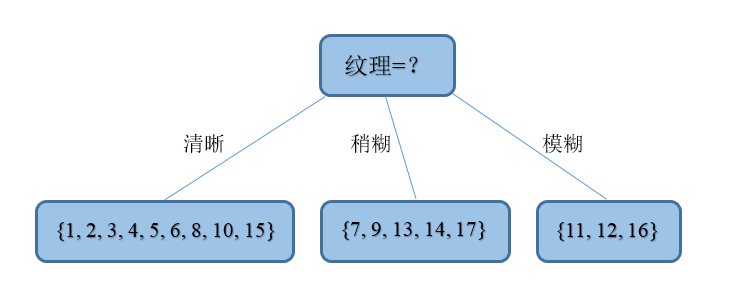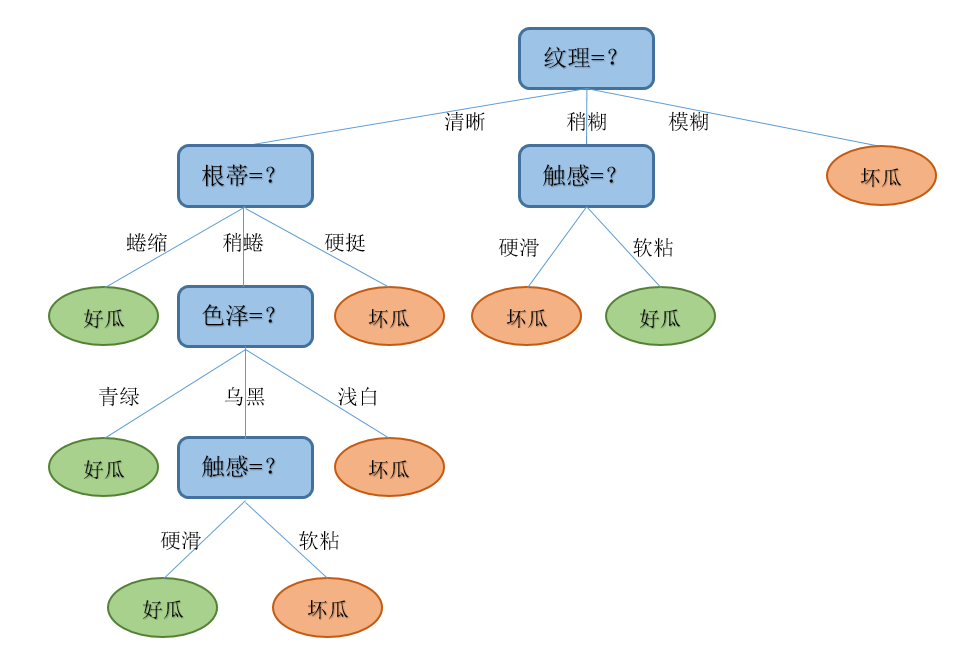2.2 õ┐Īµü»Õó×ńøŖńÄć - C4.5

0

0

，Ķ«Īń«ŚŌĆ£ń╝¢ÕÅĘŌĆØÕłåń▒╗µēĆÕĖ”µØźńÜäõ┐Īµü»Õó×ńøŖõĖ║：

Gain

(

D

,

a

)

=

H

(

D

)

ŌłÆ

Ōłæ

v

=

1

V

ŌłŻ

D

v

ŌłŻ

ŌłŻ

D

ŌłŻ

H

(

ŌłŻ

D

v

ŌłŻ

)

=

ŌłÆ

8

17

log

ŌüĪ

2

8

17

ŌłÆ

8

17

log

ŌüĪ

2

8

17

ŌłÆ

Ōłæ

v

=

1

17

1

17

├Ś

0

=

0.9975

\begin{aligned} \textrm{Gain}(D,a)&=H(D)-\sum_{v=1}^V \frac{|D_v|}{|D|}H(|D_v|) \\ &=-\frac{8}{17}\log_2\frac{8}{17}-\frac{8}{17}\log_2\frac{8}{17}-\sum_{v=1}^{17}\frac{1}{17}\times0 \\ &=0.9975 \end{aligned}

Gain

ratio

(

D

,

a

)

=

G

a

i

n

(

D

,

a

)

I

V

(

a

)

{\textrm{Gain}}_\textrm{ratio}(D,a)=\frac{\rm{Gain}(D,a)}{\rm{IV}(a)}

ÕģČõĖŁ，

IV

(

a

)

=

ŌłÆ

Ōłæ

v

=

1

V

ŌłŻ

D

v

ŌłŻ

ŌłŻ

D

ŌłŻ

log

ŌüĪ

ŌłŻ

D

v

ŌłŻ

ŌłŻ

D

ŌłŻ

=

H

(

a

)

\textrm{IV}(a)=-\sum_{v=1}^V \frac{|D_v|}{|D|}\log \frac{|D_v|}{|D|}=H(a)

H

(

Ķ¦”

µä¤

)

=

0.874

┬Ā

(

V

=

2

)

H(Ķ¦”µä¤)=0.874\ (V=2)

，

H

(

Ķē▓

µ│Į

)

=

1.580

┬Ā

(

V

=

3

)

H(Ķē▓µ│Į)=1.580\ (V=3)

，

H

(

ń╝¢

ÕÅĘ

)

=

4.088

┬Ā

(

V

=

17

)

H(ń╝¢ÕÅĘ)=4.088\ (V=17)

ŃĆé
2.3 Õ¤║Õ░╝µīćµĢ░ - CART

D

D

Gini

(

D

)

=

Ōłæ

k

=

1

ŌłŻ

Y

ŌłŻ

Ōłæ

k

ŌĆ▓

ŌēĀ

k

p

k

p

k

ŌĆ▓

=

1

ŌłÆ

Ōłæ

k

=

1

Y

p

k

2

\textrm{Gini}(D) = \sum_{k=1}^{|\mathcal{Y}|}\sum_{k^\prime\neq k}p_kp_{k^\prime}=1-\sum_{k=1}^{\mathcal{Y}}p_k^2

ńø┤Ķ¦éµØźń£ŗ，

Gini

(

D

)

\textrm{Gini}(D)

ÕÅŹµśĀõ║åõ╗ÄµĢ░µŹ«ķøå

D

D

õĖŁķÜÅµ£║µŖĮÕÅ¢õĖżõĖ¬µĀĘµ£¼，ÕģČń▒╗Õł½µĀćĶ«░õĖŹõĖĆĶć┤ńÜäµ”éńÄćŃĆéÕøĀµŁż，

Gini

(

D

)

\textrm{Gini}(D)

ĶČŖÕ░Å，ÕłÖµĢ░µŹ«ķøå

D

D

ńÜäń║»Õ║”ĶČŖķ½śŃĆé
Õ»╣ńē╣Õ«ÜÕ▒×µĆ¦

a

a

ńÜäÕ¤║Õ░╝µīćµĢ░Õ«Üõ╣ēÕ”éõĖŗ：

Gain

index

(

D

,

a

)

=

Ōłæ

v

=

1

V

ŌłŻ

D

v

ŌłŻ

ŌłŻ

D

ŌłŻ

Gini

(

D

v

)

{\textrm{Gain}}_\textrm{index}(D,a) = \sum_{v=1}^{V}\frac{|D_v|}{|D|}\textrm{Gini}(D_v)

A

A

õĖŁ，ķĆēµŗ®ķéŻõĖ¬õĮ┐ÕŠŚÕłÆÕłåÕÉÄÕ¤║Õ░╝µīćµĢ░µ£ĆÕ░ÅńÜäÕ▒×µĆ¦õĮ£õĖ║µ£Ćõ╝śÕłÆÕłåÕ▒×µĆ¦，ÕŹ│：

a

ŌłŚ

=

arg

ŌüĪ

min

ŌüĪ

a

Ōłł

A

Gain

index

(

D

,

a

)

a_\ast=\arg \min \limits_{a \in A}{{\textrm{Gain}}_\textrm{index}(D,a)}

3. Õå│ńŁ¢µĀæÕē¬µ×Ø
3.1 Õå│ńŁ¢µĀæńÜäµŹ¤Õż▒ÕćĮµĢ░
ÕłÜÕ╝ĆÕ¦ŗµłæµÅÉÕł░，Õå│ńŁ¢µĀæÕÅ»õ╗źń£ŗõĮ£µś»õĖĆń│╗ÕłŚ if-then Ķ¦äÕłÖńÜäķøåÕÉłŃĆéĶ┐ÖõĖ¬Ķ¦äÕłÖķøåÕÉłµ£ēõĖĆõĖ¬ķćŹĶ”üńÜäµĆ¦Ķ┤©：õ║Æµ¢źÕ╣ČõĖöÕ«īÕżćŃĆéµäÅµĆØÕ░▒µś»Ķ»┤，µŗ┐µØźõ╗╗µäÅõĖĆõĖ¬Õ«×õŠŗ，ķĪ║ńØĆĶ¦äÕłÖńÜäĶĄĘńé╣（µĀ╣ń╗ōńé╣）Õć║ÕÅæ，µ£Ćń╗łķāĮµ£ēõĖöÕÅ¬µ£ēõĖĆµØĪĶĘ»ÕŠäÕł░ĶŠŠµ¤ÉõĖĆõĖ¬ÕģĘõĮōńÜäÕÅČń╗ōńé╣（ÕģĘõĮōńÜäÕłåń▒╗），Õ╣ČõĖöõĖŹõ╝ÜÕć║ńÄ░Õ«×õŠŗµŚĀµ│ĢÕłåń▒╗ńÜäµāģÕåĄŃĆé

T

T

ńÜäÕÅČń╗ōńé╣õĖ¬µĢ░õĖ║

ŌłŻ

T

ŌłŻ

|T|

，

t

t

µś»µĀæ

T

T

ńÜäõĖĆõĖ¬ÕÅČń╗ōńé╣，Ķ»źÕÅČń╗ōńé╣µ£ē

N

t

N_t

õĖ¬µĀĘµ£¼ńé╣，ÕģČõĖŁ

k

k

ń▒╗ńÜäµĀĘµ£¼ńé╣µ£ē

N

t

k

N_{tk}

õĖ¬，

k

=

1

,

2

,

.

.

.

,

K

k=1,2,...,K

，

K

K

õĖ║µĀĘµ£¼ń®║ķŚ┤õĖŁńÜäµēĆÕ▒×Õłåń▒╗µĢ░ķćÅŃĆéÕÅČń╗ōńé╣

t

t

õĖŖńÜäń╗Åķ¬īńåĄ

H

t

(

T

)

H_t(T)

õĖ║

H

t

(

T

)

=

ŌłÆ

Ōłæ

k

N

t

k

N

t

log

ŌüĪ

N

t

k

N

t

H_t(T)=-\sum_k\frac{N_{tk}}{Nt}\log\frac{N_{tk}}{N_t}

k

n

k_n

µŚČ，ķéŻõ╣ł

N

t

k

n

=

N

t

N_{tk_n}=N_t

，ÕģČÕ«āńÜä

N

k

1

,

.

.

.

,

N

k

n

ŌłÆ

1

,

N

k

n

+

1

,

.

.

.

,

N

k

K

N_{k_1},...,N_{k_{n-1}},N_{k_{n+1}},...,N_{k_K}

0

0

，µ£Ćń╗ł

H

t

(

T

)

=

0

H_t(T)=0

H

t

(

T

)

H_t(T)

C

(

T

)

=

Ōłæ

t

=

1

ŌłŻ

T

ŌłŻ

N

t

H

t

(

T

)

=

ŌłÆ

Ōłæ

t

=

1

ŌłŻ

T

ŌłŻ

Ōłæ

k

=

1

K

N

t

k

log

ŌüĪ

N

t

k

N

t

C(T)=\sum_{t=1}^{|T|}N_tH_t(T)=-\sum_{t=1}^{|T|}\sum_{k=1}^K N_{tk}\log\frac{N_{tk}}{N_t}

C

(

T

)

C(T)

H

t

(

T

)

=

0

H_t(T)=0

，µ£Ćń╗łõĮ┐ÕŠŚ

C

(

T

)

=

0

C(T)=0

µ£ĆÕ░ÅŃĆé

ŌłŻ

T

ŌłŻ

|T|

C

╬▒

(

T

)

=

C

(

T

)

+

╬▒

ŌłŻ

T

ŌłŻ

C_\alpha(T)=C(T)+\alpha|T|

µØźõĮ£õĖ║õ╝śÕī¢ńÜäńø«µĀćÕćĮµĢ░，õ╣¤Õ░▒µś»µĀæńÜäµŹ¤Õż▒ÕćĮµĢ░ŃĆéÕÅéµĢ░

╬▒

\alpha

╬▒

\alpha

╬▒

\alpha

Õ”éµ×£õ╗źµ¢ćń½Āµ£ĆÕ╝ĆÕ¦ŗµĀćńż║ńÜäõĖēõĖ¬ķĆÆÕĮÆÕŁ”õ╣ĀĶ┐öÕø×µØĪõ╗ČĶ┐øĶĪīµĀæńÜäÕŁ”õ╣Ā，ķéŻõ╣łµ£ĆÕÉÄÕŁ”õ╣ĀńÜäµĀæµś»õĖĆõĖ¬õ╗ź

C

(

T

)

C(T)

õĖ║õ║åµÅÉķ½śÕå│ńŁ¢µĀæńÜäµ│øÕī¢ĶāĮÕŖø，ķ£ĆĶ”üÕ»╣µĀæĶ┐øĶĪī Õē¬µ×Ø (Pruning)，µŖŖĶ┐ćõ║Äń╗åÕłåńÜäÕÅČń╗ōńé╣（ķĆÜÕĖĖµś»µĢ░µŹ«ķćÅĶ┐ćÕ░æÕ»╝Ķć┤ÕÖ¬ÕŻ░µĢ░µŹ«ńÜäÕĮ▒ÕōŹÕó×ÕŖĀ）ÕÄ╗µÄēĶĆīÕø×ķĆĆÕł░ÕģČńłČń╗ōńé╣µł¢µø┤ķ½śńÜäń╗ōńé╣，õĮ┐ÕģČńłČń╗ōńé╣µł¢µø┤ķ½śńÜäń╗ōńé╣ÕÅśõĖ║ÕÅČń╗ōńé╣ŃĆé
3.2 Õ”éõĮĢĶ┐øĶĪīÕå│ńŁ¢µĀæÕē¬µ×Ø
3.2.1 ķóäÕē¬µ×Ø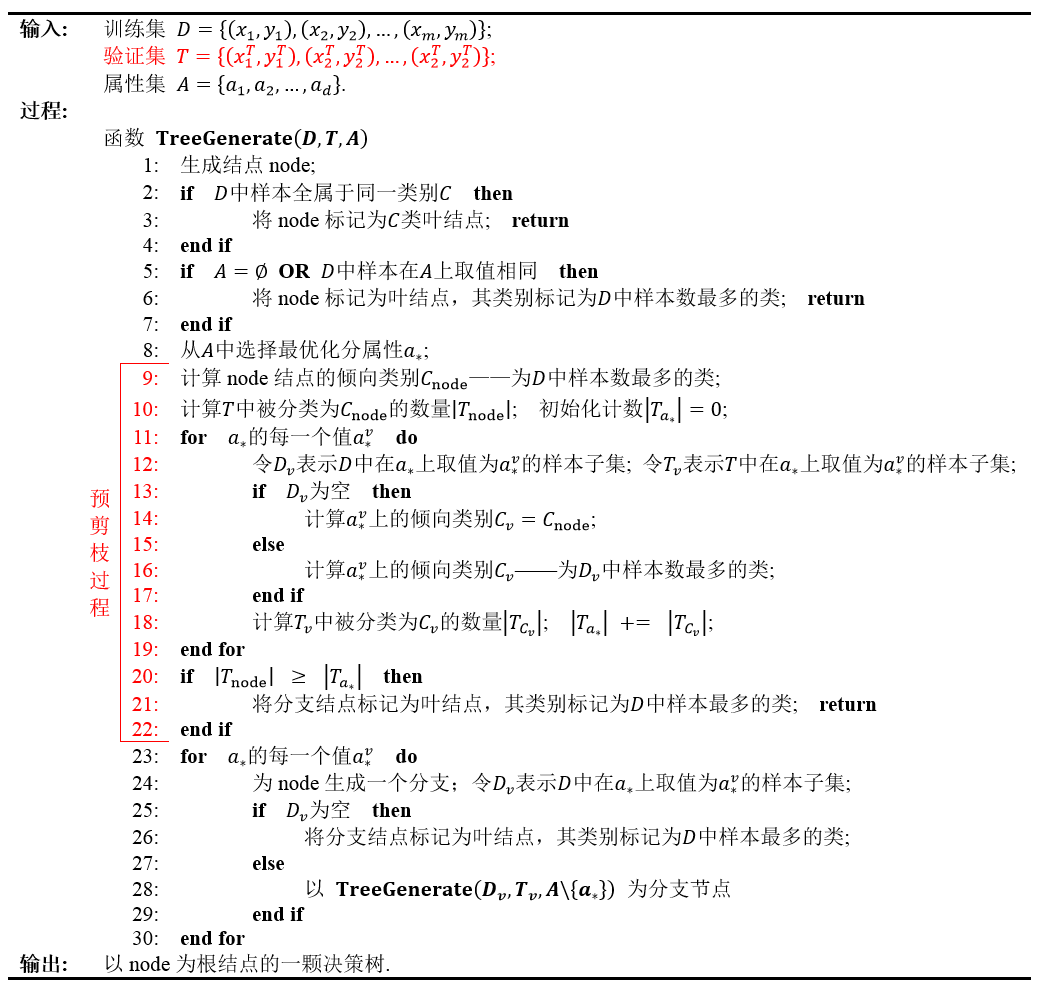3.2.2 ÕÉÄÕē¬µ×Ø

ÕÉÄÕē¬µ×ØÕłÖµś»Õģłõ╗ÄĶ«Łń╗āķøåńö¤µłÉõĖĆķóŚÕ«īµĢ┤ńÜäÕå│ńŁ¢µĀæ，ńäČÕÉÄĶć¬Õ║ĢÕÉæõĖŖÕ£░Õ»╣ķØ×ÕÅČń╗ōńé╣Ķ┐øĶĪīĶĆāÕ»¤，ĶŗźÕ░åĶ»źń╗ōńé╣Õ»╣Õ║öńÜäÕŁÉµĀæµø┐µŹóõĖ║ÕÅČń╗ōńé╣ĶāĮÕĖ”µØźµ│øÕī¢µĆ¦ĶāĮµÅÉÕŹć，ÕłÖÕ░åĶ»źÕŁÉµĀæµø┐µŹóõĖ║ÕÅČń╗ōńé╣ŃĆé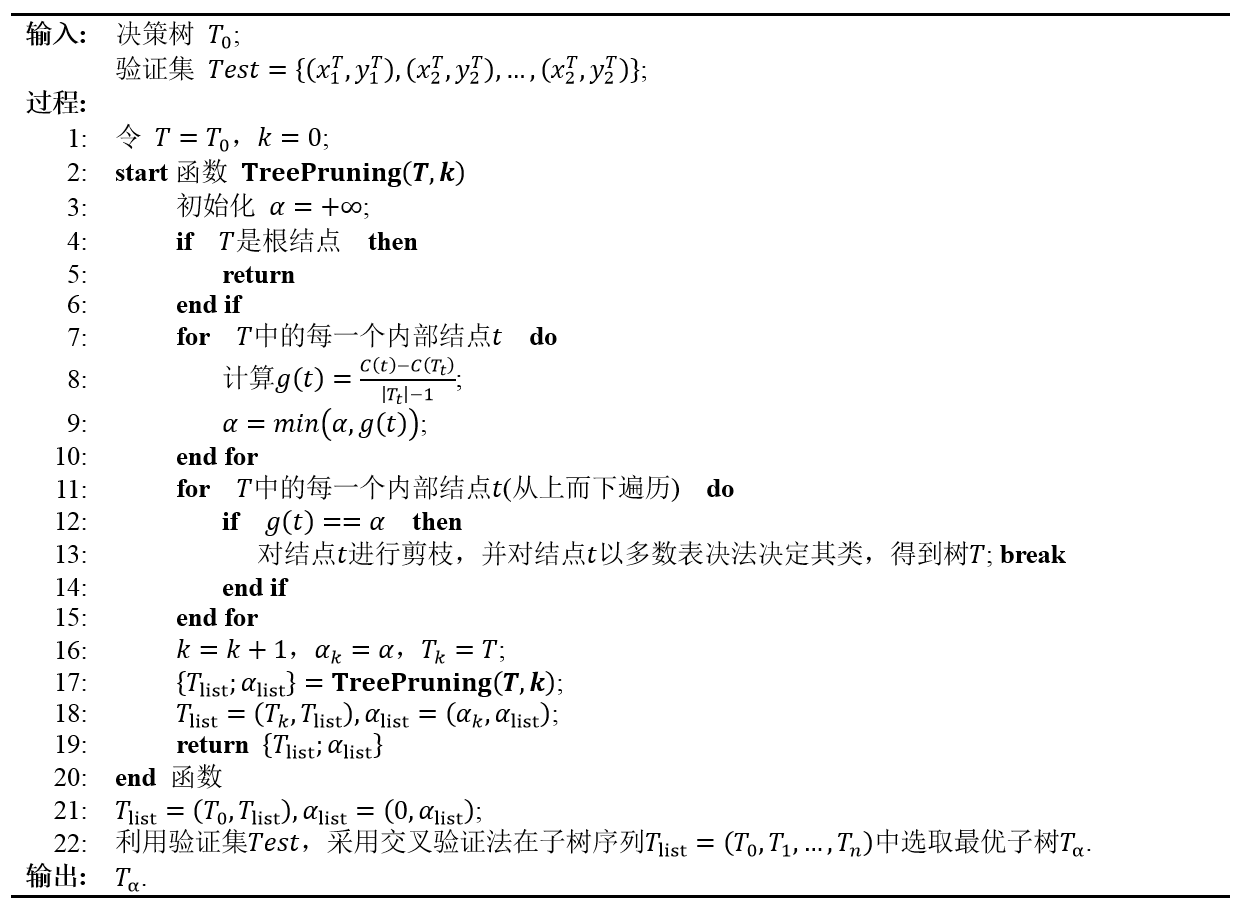T

0

T_0

t

t

，õ╗ź

t

t

õĖ║ÕŹĢń╗ōńé╣µĀæ（ń╗ōńé╣ÕŹ│õĖ║ÕÅČ，µ▓Īµ£ēÕłåµö»）ńÜäµŹ¤Õż▒ÕćĮµĢ░µś»

C

╬▒

(

t

)

=

C

(

t

)

+

╬▒

C_\alpha(t)=C(t)+\alpha

õ╗ź

t

t

õĖ║µĀ╣ń╗ōńé╣ńÜäÕŁÉµĀæ

T

t

T_t

ńÜäµŹ¤Õż▒ÕćĮµĢ░µś»

C

╬▒

(

T

t

)

=

C

(

T

t

)

+

╬▒

ŌłŻ

T

t

ŌłŻ

C_\alpha(T_t)=C(T_t)+\alpha|T_t|

ÕĮō

╬▒

=

0

\alpha=0

µł¢ÕģģÕłåÕ░ÅµŚČ，µ£ēõĖŹńŁēÕ╝Å

C

╬▒

(

T

t

)

<

C

╬▒

(

t

)

C_\alpha(T_t)<C_\alpha(t)

ÕĮō

╬▒

\alpha

Õó×Õż¦Õł░µ¤ÉõĖĆõĖ¬ÕĆ╝µŚČ，µ£ē

C

╬▒

(

T

t

)

=

C

╬▒

(

t

)

C_\alpha(T_t)=C_\alpha(t)

ÕĮō

╬▒

\alpha

ÕåŹń╗¦ń╗ŁÕó×Õż¦µŚČ，õĖŹńŁēÕ╝ÅÕÅŹÕÉæ，µēĆõ╗źÕÅ¬Ķ”ü

╬▒

=

C

(

t

)

ŌłÆ

C

(

T

t

)

ŌłŻ

T

t

ŌłŻ

ŌłÆ

1

\alpha=\frac{C(t)-C(T_t)}{|T_t|-1}

，

T

t

T_t

õĖÄ

t

t

µ£ēńøĖÕÉīńÜäµŹ¤Õż▒ÕćĮµĢ░ÕĆ╝，ĶĆī

t

t

ńÜäń╗ōńé╣µø┤Õ░æ，ÕøĀµŁż

t

t

µ»ö

T

t

T_t

µø┤ÕÅ»ÕÅ¢，Õ»╣

T

t

T_t

Ķ┐øĶĪīÕē¬µ×ØŃĆé
Ķ┐ÖõĖ¬õĮŹńĮ«Õ«╣µśōĶ«®õ║║ń¢æµāæ，ķĆ╗ĶŠæõĖŖĶ«®õ║║õĖŹµś»ÕŠłÕ«╣µśōµā│µśÄńÖĮ，ķ£ĆĶ”üÕżÜń£ŗÕćĀķüŹŃĆéÕģČÕ«×Õ«āńÜäķĆ╗ĶŠæµś»Ķ┐ÖµĀĘńÜä：

╬▒

\alpha

ÕĆ╝ĶĪĪķćÅõ║åµŹ¤Õż▒ÕćĮµĢ░õĖŁÕÅČń╗ōńé╣µĢ░ķćÅńÜäµØāķćŹ，

╬▒

\alpha

╬▒

\alpha

ÕÅ»õ╗źń£ŗõĮ£õĖĆõĖ¬ń│╗µĢ░，õĖŹÕÉīńÜä

╬▒

\alpha

Ķ┐ÖõĖ¬µ£ēńé╣ń▒╗õ╝╝õ║ÄÕ»╣õĖĆõĖ¬ÕćĮµĢ░ńÜäµ│░ÕŗÆń║¦µĢ░Õ▒ĢÕ╝Ć，ĶĆī

╬▒

\alpha

ÕĆ╝µÄ¦ÕłČńØĆÕ▒ĢÕ╝ĆńÜäµ¼ĪµĢ░ķĪ╣，ĶČŖÕ░ÅńÜäÕĆ╝Õ▒ĢÕ╝ĆńÜäµ¼ĪµĢ░ķĪ╣ĶČŖÕżÜ（ÕŠĆÕø×µöČń╝®ńÜäķ½śµ¼ĪķĪ╣ĶČŖÕ░æ）ŃĆéÕøĀõĖ║Õå│ńŁ¢µĀæńÜäń╗ōńé╣õĖ¬µĢ░µś»µ£ēķÖÉńÜä，Õ»╣Õ║öÕł░

╬▒

\alpha

ńÜäÕĆ╝µØźĶ»┤õ╣¤µś»µ£ēķÖÉńÜäŃĆéõĖŖĶ┐░µ▒é

╬▒

\alpha

╬▒

\alpha

Ķ┐Öķćīµ£ēõĖĆõĖ¬ń¢æµāæÕ░▒µś»，µīēńģ¦Ķ┐ÖµĀĘńÜäķĆ╗ĶŠæµØźĶÄĘÕŠŚńÜä

╬▒

list

\alpha_\textrm{list}

ń£¤ńÜäµ╗ĪĶČ│ķĆÆÕó×ķĪ║Õ║ÅÕÉŚ？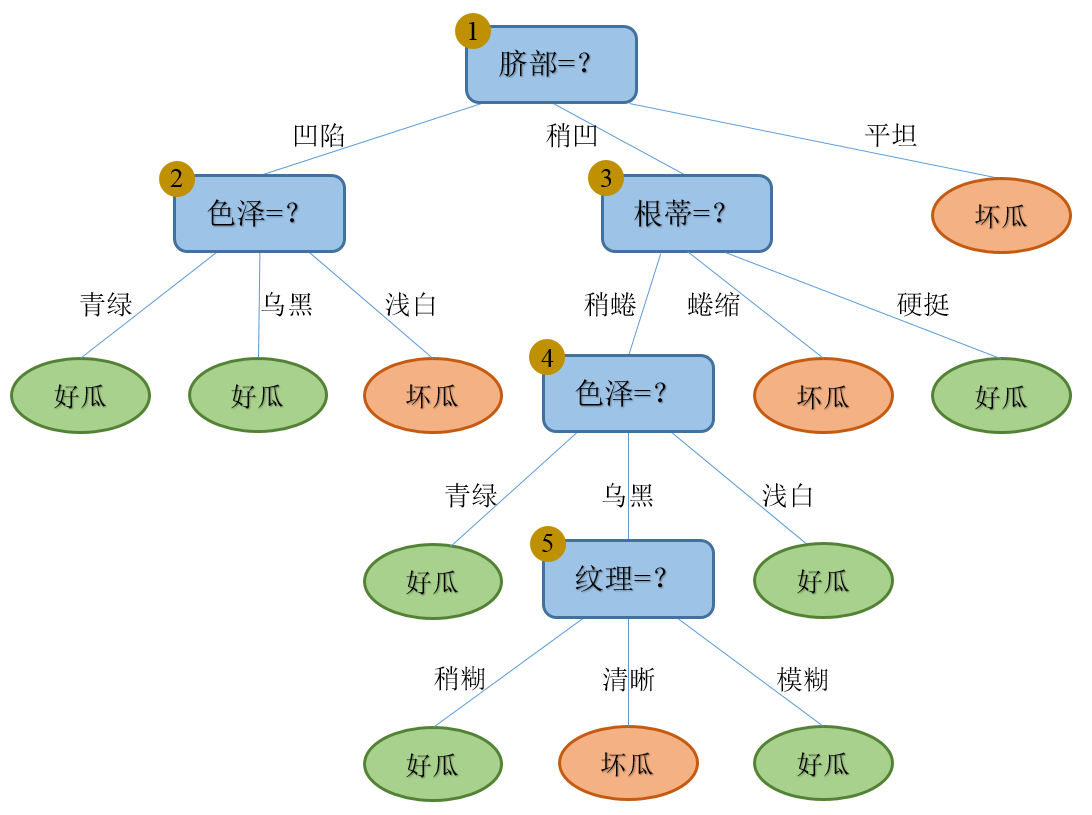C

(

T

)

C(T)

ÕÆī

C

╬▒

(

T

)

C_\alpha(T)

ńÜäÕ«Üõ╣ēŃĆé
1. ń¼¼õĖĆÕ▒éķĆÆÕĮÆ： µĀæ

T

0

=

{

1

,

2

,

3

,

4

,

5

}

T_0=\lbrace 1,2,3,4,5\rbrace

，ÕģČÕ£©Ķ«Łń╗āķøåµĢ░µŹ«õĖŖńÜäµē¦ĶĪīµāģÕåĄĶ¦üõĖŗÕøŠ：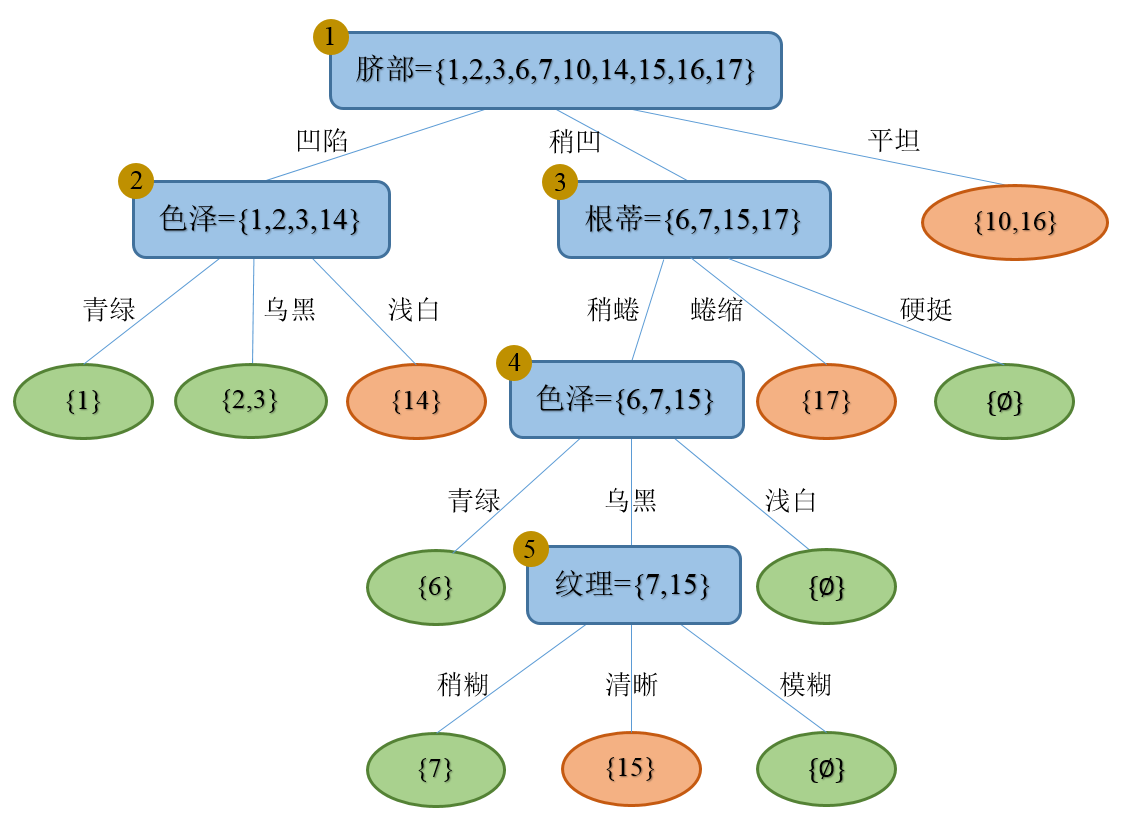Õ»╣ÕģČµ»ÅõĖĆõĖ¬Õåģķā©ń╗ōńé╣Ķ«Īń«ŚÕģČÕē¬µ×ØÕÉÄńÜäµĢ┤õĮōµĀæńÜäµŹ¤Õż▒ÕćĮµĢ░ÕćÅÕ░æń©ŗÕ║”

g

(

t

)

g(t)

ŃĆé õ╗źń╗ōńé╣

5

5

õĖ║õŠŗ，õĖĆõŠŗÕźĮõĖĆõŠŗÕØÅ，

C

(

5

)

=

ŌłÆ

Ōłæ

t

=

1

1

Ōłæ

k

Ōłł

(

ÕźĮ

ŃĆü

ÕØÅ

)

N

t

k

log

ŌüĪ

2

N

t

k

N

t

=

ŌłÆ

1

├Ś

log

ŌüĪ

2

(

1

/

2

)

ŌłÆ

1

├Ś

log

ŌüĪ

2

(

1

/

2

)

)

=

2

;

C

(

T

5

)

=

ŌłÆ

Ōłæ

t

=

1

3

Ōłæ

k

Ōłł

(

ÕźĮ

ŃĆü

ÕØÅ

)

N

t

k

log

ŌüĪ

2

N

t

k

N

t

=

ŌłÆ

(

1

├Ś

log

ŌüĪ

2

(

1

/

1

)

+

0

├Ś

log

ŌüĪ

2

(

0

/

1

)

)

ŌłÆ

(

0

├Ś

log

ŌüĪ

2

(

0

/

1

)

+

1

├Ś

log

ŌüĪ

2

(

1

/

1

)

)

ŌłÆ

0

=

0

;

ŌłŻ

T

5

ŌłŻ

=

3

;

g

(

5

)

=

C

(

5

)

ŌłÆ

C

(

T

5

)

ŌłŻ

T

5

ŌłŻ

ŌłÆ

1

=

2

ŌłÆ

0

3

ŌłÆ

1

=

1.

\begin{aligned} C(5)&=-\sum_{t=1}^1\sum_{k \in (ÕźĮŃĆüÕØÅ)}N_{tk}\log_2\frac{N_{tk}}{N_t} \\ &=-1\times\log_2(1/2)-1\times\log_2(1/2)) \\ &=2; \\ C(T_5)&=-\sum_{t=1}^3\sum_{k \in (ÕźĮŃĆüÕØÅ)}N_{tk}\log_2\frac{N_{tk}}{N_t} \\ &=-\left(1\times\log_2(1/1)+0\times\log_2(0/1)\right)-\left(0\times\log_2(0/1)+1\times\log_2(1/1)\right)-0 \\ &=0; \\ |T_5|&=3; \\ g(5)&=\frac{C(5)-C(T_5)}{|T_5|-1}=\frac{2-0}{3-1}=1. \end{aligned}

ÕÉīńÉå，ÕÅ»õ╗źĶ«Īń«ŚÕć║:

g

(

4

)

=

C

(

4

)

ŌłÆ

C

(

T

4

)

ŌłŻ

T

4

ŌłŻ

ŌłÆ

1

=

2.7549

ŌłÆ

0

5

ŌłÆ

1

=

0.6887

g

(

3

)

=

C

(

3

)

ŌłÆ

C

(

T

3

)

ŌłŻ

T

3

ŌłŻ

ŌłÆ

1

=

4

ŌłÆ

0

7

ŌłÆ

1

=

0.5714

g

(

2

)

=

C

(

2

)

ŌłÆ

C

(

T

2

)

ŌłŻ

T

2

ŌłŻ

ŌłÆ

1

=

3.2451

ŌłÆ

0

3

ŌłÆ

1

=

1.6226

g

(

1

)

=

C

(

1

)

ŌłÆ

C

(

T

1

)

ŌłŻ

T

1

ŌłŻ

ŌłÆ

1

=

10

ŌłÆ

0

11

ŌłÆ

1

=

1

\begin{aligned} g(4)&=\frac{C(4)-C(T_4)}{|T_4|-1}=\frac{2.7549-0}{5-1}=0.6887 \\ g(3)&=\frac{C(3)-C(T_3)}{|T_3|-1}=\frac{4-0}{7-1}=0.5714 \\ g(2)&=\frac{C(2)-C(T_2)}{|T_2|-1}=\frac{3.2451-0}{3-1}=1.6226 \\ g(1)&=\frac{C(1)-C(T_1)}{|T_1|-1}=\frac{10-0}{11-1}=1 \end{aligned}

µ»öĶŠāõ╣ŗÕÉÄÕÅæńÄ░，µ£ĆÕ░ÅńÜäµś»

g

(

3

)

g(3)

，Õ»╣Õ║öńÜäń╗ōńé╣µś»

t

(

3

)

t(3)

，Õ»╣ÕģČĶ┐øĶĪīÕē¬µ×ØÕÉÄÕŠŚÕł░µ¢░ńÜäµĀæ

T

1

T_1

Õ£©Ķ«Łń╗āķøåµĢ░µŹ«õĖŖńÜäµē¦ĶĪīµāģÕåĄĶ¦üõĖŗÕøŠ：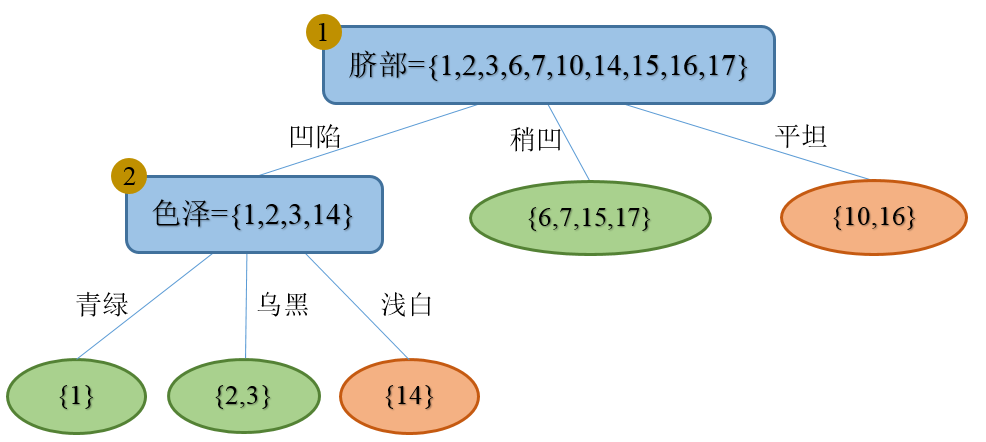2. ń¼¼õ║īÕ▒éķĆÆÕĮÆ： õ╗źµĀæ

T

1

=

{

1

,

2

}

T_1=\lbrace 1,2\rbrace

õĖ║µ¢░ńÜäĶŠōÕģź，ķćŹÕżŹń¼¼õĖĆÕ▒éķĆÆÕĮÆõĖŁńÜäĶ«Īń«ŚµŁźķ¬ż：

g

(

2

)

=

C

(

2

)

ŌłÆ

C

(

T

2

)

ŌłŻ

T

2

ŌłŻ

ŌłÆ

1

=

3.2451

ŌłÆ

0

3

ŌłÆ

1

=

1.6226

g

(

1

)

=

C

(

1

)

ŌłÆ

C

(

T

1

)

ŌłŻ

T

1

ŌłŻ

ŌłÆ

1

=

10

ŌłÆ

0

5

ŌłÆ

1

=

2.5

\begin{aligned} g(2)&=\frac{C(2)-C(T_2)}{|T_2|-1}=\frac{3.2451-0}{3-1}=1.6226 \\ g(1)&=\frac{C(1)-C(T_1)}{|T_1|-1}=\frac{10-0}{5-1}=2.5 \end{aligned}

µ»öĶŠāõ╣ŗÕÉÄÕÅæńÄ░，µ£ĆÕ░ÅńÜäµś»

g

(

2

)

g(2)

，Õ»╣Õ║öńÜäń╗ōńé╣µś»

t

(

2

)

t(2)

，Õ»╣ÕģČĶ┐øĶĪīÕē¬µ×ØÕÉÄÕŠŚÕł░µ¢░ńÜäµĀæ

T

2

T_2

Õ£©Ķ«Łń╗āķøåµĢ░µŹ«õĖŖńÜäµē¦ĶĪīµāģĶ¦üõĖŗÕøŠ: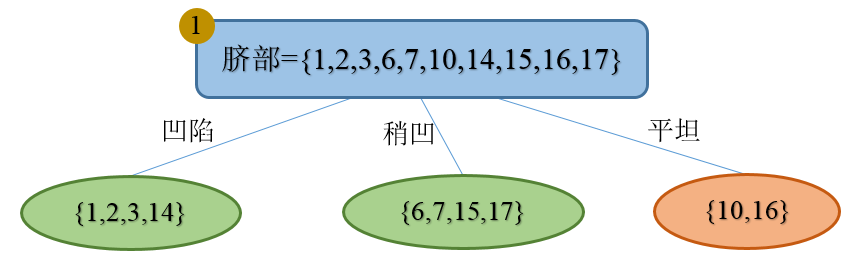3. ń¼¼õĖēÕ▒éķĆÆÕĮÆ： õ╗źµĀæ

T

2

=

{

1

}

T_2=\lbrace 1\rbrace

õĖ║µ¢░ńÜäĶŠōÕģź，ķćŹÕżŹń¼¼õĖĆÕ▒éķĆÆÕĮÆõĖŁńÜäĶ«Īń«ŚµŁźķ¬ż：

g

(

1

)

=

C

(

1

)

ŌłÆ

C

(

T

1

)

ŌłŻ

T

1

ŌłŻ

ŌłÆ

1

=

10

ŌłÆ

0

3

ŌłÆ

1

=

5

g(1)=\frac{C(1)-C(T_1)}{|T_1|-1}=\frac{10-0}{3-1}=5

ńÄ`